# Quantum Well Semiconductor Lasers

This is a continuation from the previous tutorial - diverse applications of coupled-cavity semiconductor lasers.

## 1. Energy Levels

A double-heterostructure laser consists of an active layer sandwiched between two higher-gap cladding layers. The active-layer thickness is typically in the range of 0.1-0.3 μm.

In recent years, double-heterostructure lasers with an active-layer thickness of ~ 10 nm have been fabricated. The carrier (electron or hole) motion normal to the active layer in these structures is restricted. As a result, the kinetic energy of the carriers moving in that direction is quantized into discrete energy levels similar to the well-known quantum-mechanical problem of the one-dimensional potential well, and hence these lasers are called quantum-well lasers.

When the thickness of the active region (or any low-gap semiconductor layer confined between higher-gap semiconductors) becomes comparable to the de Broglie wavelength ($$\lambda=h/p$$), quantum-mechanical effects are expected to occur. These effects are observed in the absorption and emission characteristics (including lasing action) and transport characteristics (including phenomena such as tunneling).

The optical characteristics of quantum-well double-heterostructure lasers were initially studied in 1974. Since then, AlGaAs quantum-well lasers have been extensively studied. Quantum effects also occur on surface states of a semiconductor or other types of surfaces. These effects have been discussed in several review articles.

A carrier (electron or hole) in a double heterostructure is confined within a three-dimensional potential well. The energy levels of such carriers are obtained by separating the system Hamiltonian into three parts, corresponding to the kinetic energies in the $$x$$, $$y$$, and $$z$$ directions.

When the thickness of the active layer ($$L_z$$) is comparable to the de Broglie wavelength, the kinetic energy corresponding to the carrier motion along the $$z$$ direction is quantized.

Along the $$x$$ and $$y$$ directions, the energy levels form a continuum of states given by

$\tag{9-1-1}E=(\hbar^2/2m)(k_x^2+k_y^2)$

where $$m$$ is the effective mass of the carrier and $$k_x$$ and $$k_y$$ are the wavevector components along the $$x$$ and $$y$$ directions, respectively.

Thus the electrons or holes in a quantum well may be viewed as forming a two-dimensional Fermi gas.

The energy levels in the $$z$$ direction are obtained by solving the Schrödinger equation for a one-dimensional potential well given by

\tag{9-1-2}\begin{align}&E\psi=-\frac{\hbar^2}{2m}\frac{\text{d}^2\psi}{\text{d}z^2}\qquad&\text{inside the well }(0\le{z}\le{L_z})\\&E\psi=-\frac{\hbar^2}{2m}\frac{\text{d}^2\psi}{\text{d}z^2}+V\psi\qquad&\text{outside the well }(z\ge{L_z;z\le0})\end{align}

where $$\psi$$ is the Schrödinger wave function and $$V$$ is the depth of the potential well.

For the limiting case of an infinitely deep well, the energy levels and wave functions are

$\tag{9-1-3a}E_n=\frac{\hbar^2}{2m}\left(\frac{n\pi}{L_z}\right)^2$

$\tag{9-1-3b}\psi_n=\begin{cases}A\sin\frac{n\pi{z}}{L_z}\qquad\text{inside the well}\\0\qquad\qquad\qquad\text{outside the well}\end{cases}$

where $$n$$ is a positive integer and $$A$$ is a normalization constant.

For very large $$L_z$$, Equation (9-1-3) yields a continuum of states and the system no longer exhibits quantum effects.

For a finite well, the energy levels and wave function can be obtained from Equation (9-1-2) by using the boundary conditions that $$\psi$$ and $$\text{d}\psi/\text{d}z$$ are continuous at the interfaces $$z=0$$ and $$z=L_z$$. The solution is

$\tag{9-1-4}\psi=\begin{cases}A\exp(k_1z)\qquad\quad(z\le0)\\B\sin(k_2z+\delta)\qquad(0\le{z}\le{L_z})\\C\exp(-k_1z)\qquad\quad(z\ge{L_z})\end{cases}$

where

$\tag{9-1-5}k_1=\left[\frac{2m(V-E)}{\hbar^2}\right]^{1/2}\qquad{k_2}=\left(\frac{2mE}{\hbar^2}\right)^{1/2}$

The quantities $$A$$, $$B$$, $$C$$ and $$\delta$$ are constants.

Using the boundary conditions stated above, the following eigenvalue equation is obtained:

$\tag{9-1-6}\tan(k_2L_z)=k_1/k_2$

The eigenvalue equation is numerically solved to yield the energy levels $$E_n$$ of a particle in a potential well.

Figure 9-1 shows the calculated energy levels of the bound states as a function of the well depth.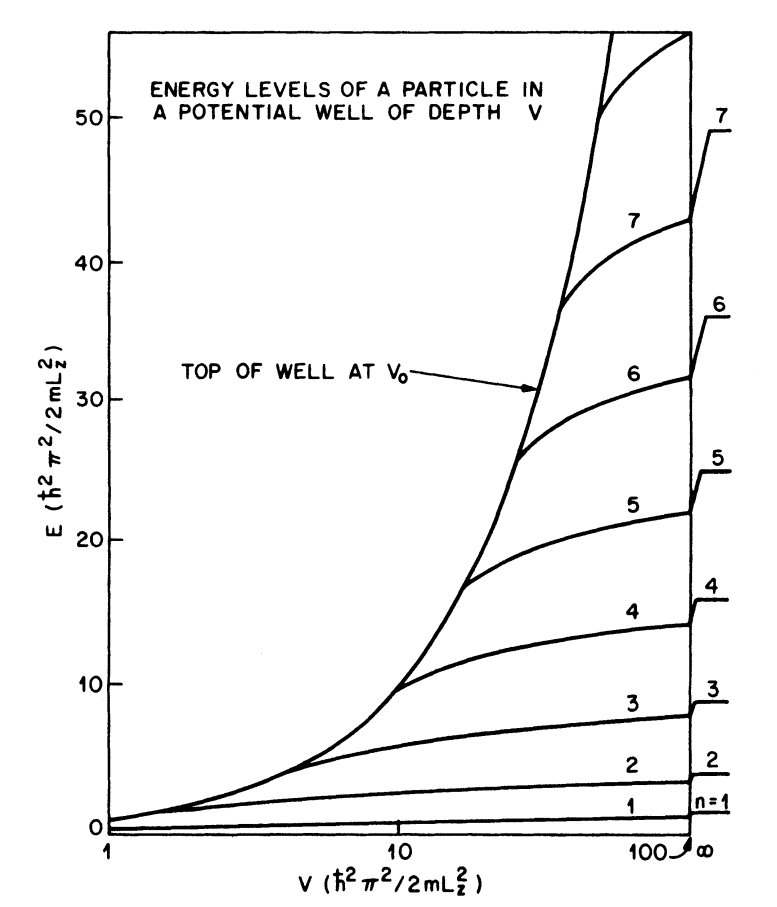Figure 9-1.  The dependence of the bound-state energy levels $$E$$ on the depth of the potential well $$V$$. The plot is given in reduced energy units, $$\hbar^2\pi^2/2mL_z^2$$. The curve represents the top of the well ($$E=V_0$$). Energy levels for an infinite potential well are shown on the extreme right.

The potential well for electrons and holes in a double heterostructure depends on the materials involved. The following values for the conduction-band ($$\Delta{E}_\text{c}$$) and valence-band ($$\Delta{E}_\text{v}$$) discontinuities are found experimentally for GaAs-AlGaAs double heterostructures:

$\tag{9-1-7a}\Delta{E}_\text{c}/\Delta{E}=0.67\pm0.01$

$\tag{9-1-7b}\Delta{E}_\text{v}/\Delta{E}=0.33\pm0.01$

where $$\Delta{E}$$ is the band-gap difference between the confining and active layers.

A knowledge of $$\Delta{E}_\text{c}$$ and $$\Delta{E}_\text{v}$$ is necessary in order to accurately calculate the energy levels.

For an InGaAsP-InP double heterostructure, the values obtained are

$\tag{9-1-8a}\Delta{E}_\text{c}/\Delta{E}=0.39\pm0.01$

$\tag{9-1-8b}\Delta{E}_\text{v}/\Delta{E}=0.61\pm0.01$

Taking into account discrete states along the $$z$$ direction and continuous states along the $$x$$ and $$y$$ directions, the energy eigenvalues for a particle confined in the quantum well are

$\tag{9-1-9}E(n,k_x,k_y)=E_n+\frac{\hbar^2}{2m_n^*}(k_x^2+k_y^2)$

where $$E_n$$ is the $$n$$th confined-particle energy level for carrier motion normal to the well and $$m_n^*$$ is the effective mass for this level.

Figure 9-2 shows schematically the energy levels $$E_n$$ of electrons and holes confined within a quantum well.

The confined-particle energy levels $$E_n$$ are denoted by $$E_\text{1c}$$, $$E_\text{2c}$$, and $$E_\text{3c}$$ for electrons; $$E_\text{1hh}$$, $$E_\text{2hh}$$, and $$E_\text{3hh}$$ for heavy holes; and $$E_\text{1lh}$$ and $$E_\text{2lh}$$ for light holes.

These quantities can be calculated by solving the eigenvalue equation (9-1-6) for a given potential barrier ($$\Delta{E}_\text{c}$$ or $$\Delta{E}_\text{v}$$).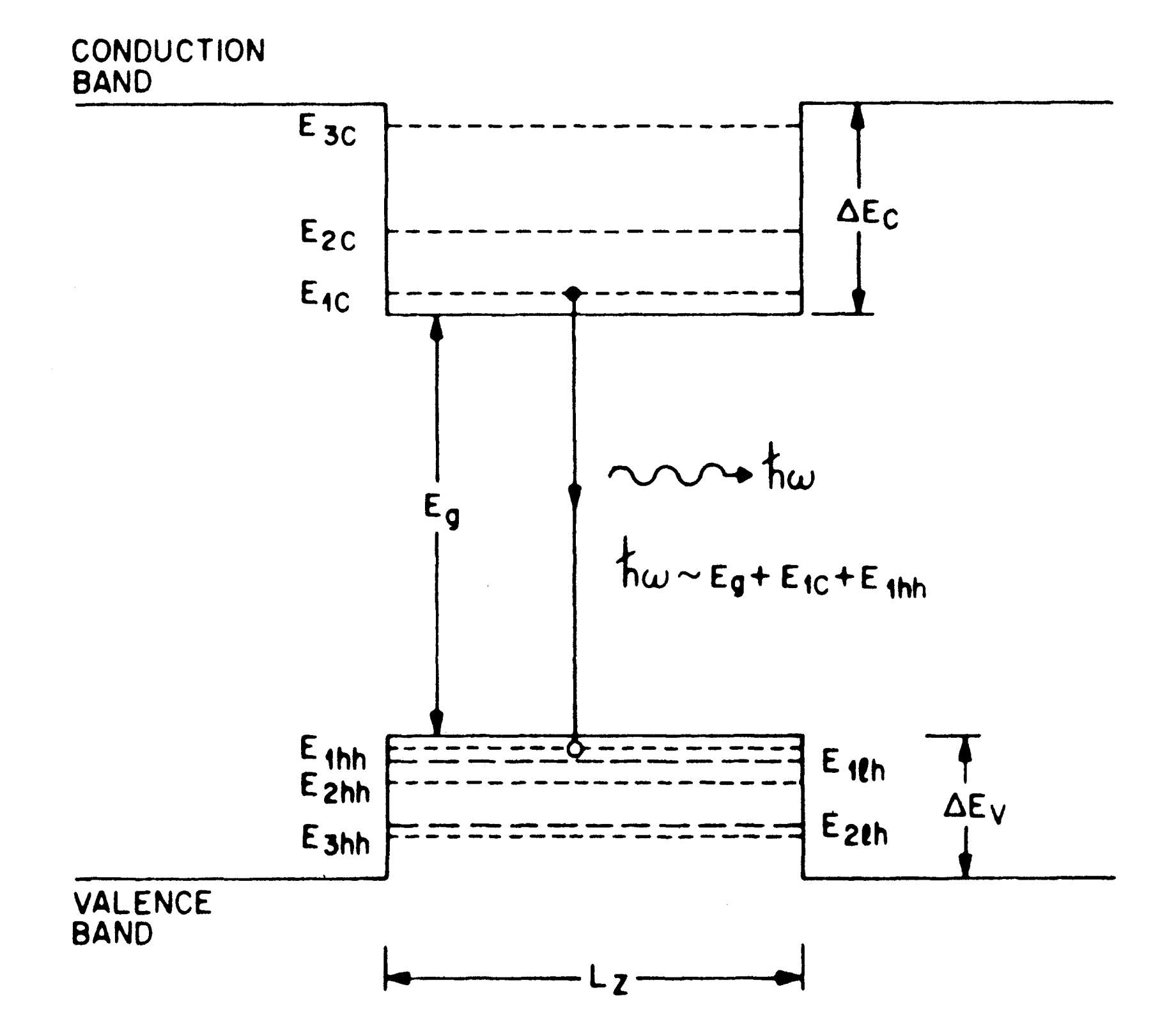Figure 9-2.  Schematic representation of the confined-particle energy levels of electrons, heavy holes (short dashes), and light holes (long dashes) in a quantum well.

## 2. Density of States

In a quantum-well structure, the kinetic energy of confined carriers (electrons or holes) for motion normal to the well ($$z$$ direction) is quantized into discrete energy levels. This modifies the density of states from the well-known three-dimensional case [see Equation (3-2-26) in the radiative recombination in semiconductors tutorial].

Using the principle of box quantization for kinetic energies along the $$x$$ and $$y$$ directions, the number of electron states per unit area in the $$x-y$$ plane for the $$i$$th subband, within an energy interval $$\text{d}E$$, is given by

$\tag{9-2-1}D_i(E)\text{d}E=2\frac{\text{d}^2\mathbf{k}}{(2\pi)^2}$

where the factor $$2$$ arises from the two spin states and $$\mathbf{k}=(k_x,k_y)$$ is the momentum vector.

Using the parabolic-band approximation, namely, $$E=\hbar^2k^2/2m_{\text{c}i}$$ [refer to Equation (3-2-1a) in the radiative recombination in semiconductors tutorial], and the relation $$\text{d}^2\mathbf{k}=2\pi{k}\text{d}k$$, we obtain

$\tag{9-2-2}D_i=\frac{m_{\text{c}i}}{\pi\hbar^2}$

where $$m_{\text{c}i}$$ is the effective mass of the electrons in the $$i$$th subband of the conduction band.

Thus the density of states per unit volume is given by

$\tag{9-2-3}g_{\text{c}i}=\frac{D_i}{L_z}=\frac{m_{\text{c}i}}{\pi\hbar^2L_z}$

A similar equation holds for holes in the valence band.

The density of states for the three-dimensional case [from Equation (3-2-26) in the radiative recombination in semiconductors tutorial], rewritten here for the sake of comparison, is

$\tag{9-2-4}\rho_\text{c}(E)=4\pi\left(\frac{2m_\text{c}}{h^2}\right)^{3/2}E^{1/2}$

A comparison of Equations (9-2-3) and (9-2-4) shows that the density of states in a quantum well is independent of the carrier energy.

Figure 9-3 shows the modification of the density of states in a quantum well. This modification can significantly alter the recombination rates in a quantum-well double heterostructure compared with those for a regular double heterostructure as discussed in the next section.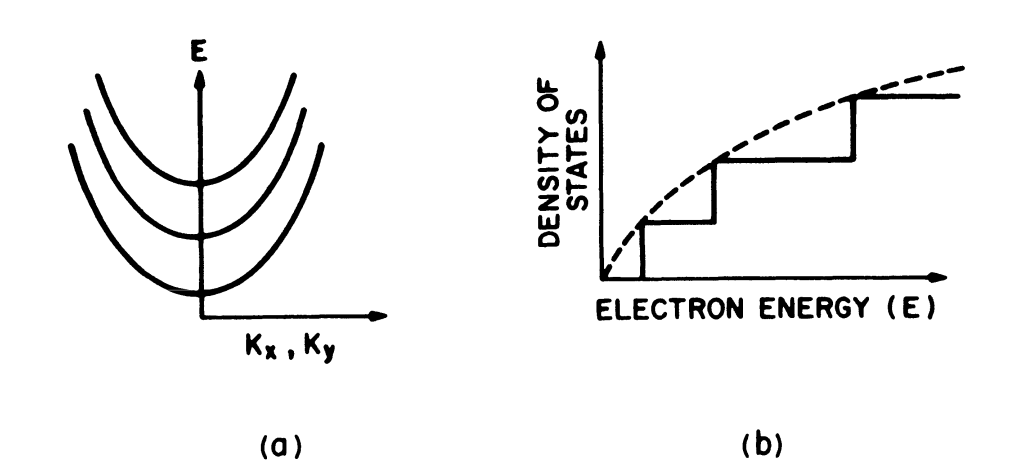Figure 9-3.  (a) Schematic representation of the two-dimensional parabolic subbands. (b) Schematic representation of the density of states in a quantum well. Dashed curve shows the density of states in a bulk semiconductor.

The simple model for the density of states just described neglects the effect of collisions between carriers that would broaden the discrete energy levels in the $$z$$ direction.

A necessary condition for the validity of the model is that the collisional broadening be small relative to the separation between discrete levels. If we use Equation (9-1-3a) to obtain the level separation $$E_2-E_1$$ between the two lowest levels, the condition is

$\tag{9-2-5}\frac{h}{2\pi\tau}\ll\frac{3h^2}{8m_\text{c}L_z^2}$

where $$\tau$$ is the electron-electron scattering time.

The term on the right-side of the equation gives the energy separation between the first two levels for an infinite square well.

It is experimentally determined that $$\tau\lt0.5\text{ ps}$$ for bulk GaAs. Using $$\tau=0.5\text{ ps}$$ and $$m_\text{c}=0.071m_0$$ (for GaAs), we get from Equation (9-2-5) that $$L_z\ll110\text{ nm}$$.

This estimate shows that Equation (9-2-3) for the density of states is a good approximation for a well thickness that is less than ~ 30 nm.

If $$n$$ is the number of electrons in the conduction band, the Fermi level can be obtained from

$\tag{9-2-6}n=\sum_i\displaystyle\int\limits_{E_{i0}}^\infty{g_{\text{c}i}}f_\text{c}(E_i)\text{d}E_i$

where

$f_\text{c}(E_i)=\frac{1}{1+\exp[(E_i-E_\text{fc})/k_\text{B}T]}$

is the Fermi function, $$E_\text{fc}$$ is the quasi-Fermi energy in the conduction band, and $$E_i$$ is the confined-particle energy level of the $$i$$th subband.

The summation is performed over all subbands in the conduction band. Since $$g_{\text{c}i}$$ does not depend on $$E_i$$, the integration is readily performed to obtain

$\tag{9-2-7}n=k_\text{B}T\sum_i{g}_{\text{c}i}\ln\left[1+\exp\left(\frac{E_\text{fc}-E_{i0}}{k_\text{B}T}\right)\right]$

Using the Boltzmann approximation and assuming that only one subband is occupied, Equation (9-2-7) becomes

$\tag{9-2-8}n=N_\text{c}\exp\left(\frac{E_\text{fc}}{k_\text{B}T}\right)$

where $$N_\text{c}=g_\text{c}k_\text{B}T$$ and $$E_\text{fc}$$ is measured from the bottom of the subband at $$E_{i0}$$.

The Fermi factor under the same approximation takes the form

$\tag{9-2-9}f_\text{c}(E)\approx\exp\left(\frac{E_\text{fc}-E}{k_\text{B}T}\right)\approx\frac{n}{N_\text{c}}\exp\left(-\frac{E}{k_\text{B}T}\right)$

Similar relations hold for holes in the valence band.

## 3. Experimental Observation of Confined States

The existence of confined-particle states was demonstrated using optical  absorption measurements.

Figure 9-4 shows a schematic diagram of the sample used.Figure 9-4.  Schematic of the multilayer sample used for optical absorption measurements. Middle drawing shows the variation in the valence- and conduction-band edges caused by the alternating GaAs and AlGaAs layers.

The samples were grown by molecular beam epitaxy and had up to 100 alternate layers of GaAs and AlGaAs (with a total GaAs thickness of ~ 400 nm) in order to provide sufficient absorption.

The measured absorption spectra at 2 K are shown in Figure 9-5.

The direct band gap of GaAs is 1.5192 eV at 2 K, and that of the cladding layer used (AlGaAs) is 1.75 eV. Thus the absorption peaks observed between these two energies are due to confined-particle discrete states and correspond to transitions between the valence band and various subbands within the conduction band.Figure 9-5.  Measured absorption spectra at 2 K of GaAs layers 400-, 21-, and 14-nm thick sandwiched between Al0.2Ga0.8As barrier layers. Multiple absorption peaks correspond to transitions between different subbands arising from quantum-size effects.

Temkin et al. have reported the measurements of absorption spectra of a 50-well InGaAs-InP sample with a well thickness of 12.5 nm. Figure 9-6 shows the measured data.

The sample was prepared by gas-source molecular-beam epitaxy (MBE) [refer to the what is molecular-beam epitaxy (MBE) tutorial. The transmission dips correspond to absorption peaks caused by transitions between various subbands. The observed shift in the absorption peaks with temperature occurs because band gap varies with temperature.Figure 9-6.  Absorption spectra of a sample with 50 InGaAs wells approximately 12.5-nm thick at various temperatures.

The lasing action usually occurs on the transition from $$E_\text{1c}$$ to $$E_\text{1hh}$$, i.e., the transition between the lowest conduction-band subband and the highest heavy-hole subband (see Figure 9-2).

One important characteristic of a quantum-well structure is that the lasing wavelength can be easily varied by varying the thickness of the well. This was clearly demonstrated for an InGaAs-InP quantum-well double heterostructure.

Figure 9-7 shows the experimental results for the optical spectra with three different well thicknesses obtained by optical pumping of the InGaAs-InP sample using a Nd-YAG laser ($$\lambda=1.06$$ μm). The wavelength shift of the main peak corresponds to the change in the lowest energy level of a quantum well with the well thickness.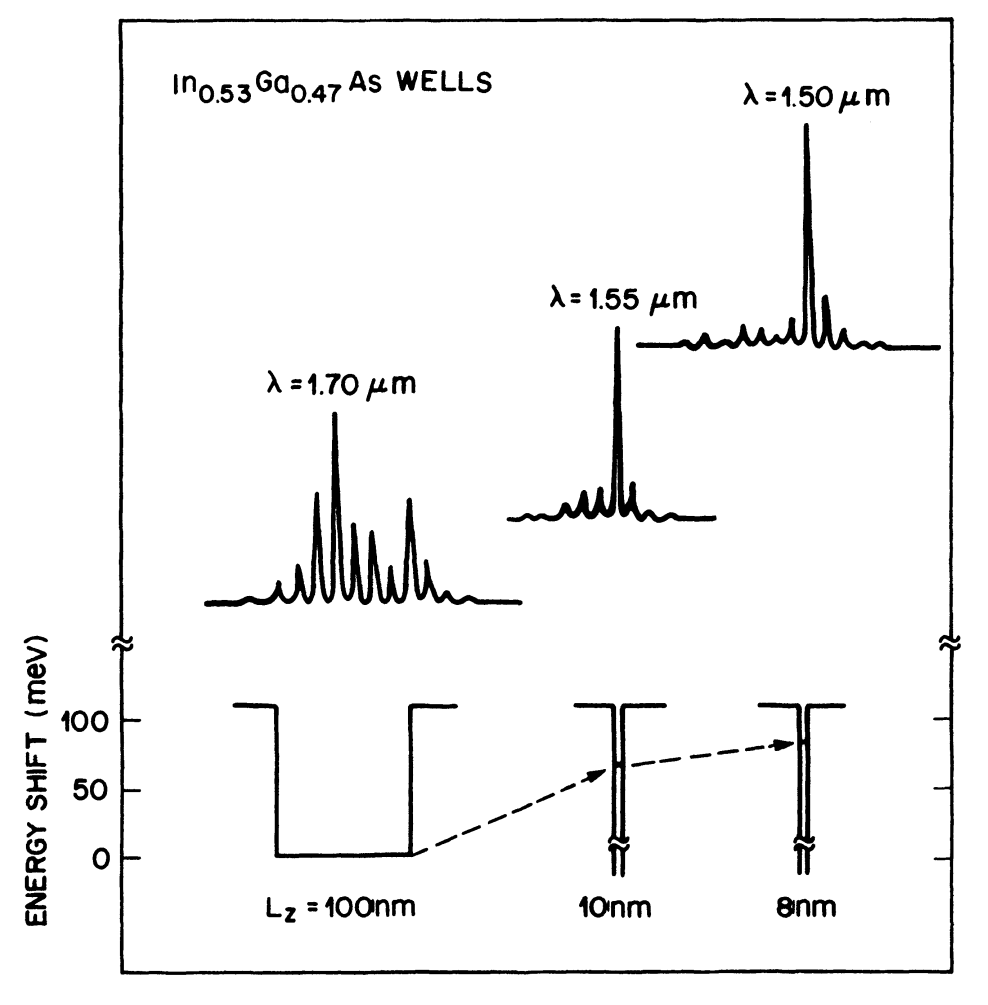Figure 9-7.  Longitudinal-mode spectra for three well thicknesses of an InGaAs-InP multiquantum-well structure. The wavelength shift corresponds to the energy-level shift of the quantum well (dashed lines).

The radiative recombination rate is obtained by calculating the two-dimensional analog of the results presented in the radiative recombination in semiconductors tutorial for bulk material.

A complete calculation of the radiative recombination rate would include summation over all possible transitions between the various subbands in the conduction and valence bands.

Before doing this calculation, we first consider a particular transition, namely, the transition from the lowest level in the conduction band (level $$\text{1c}$$) to the lowest level in the heavy-hole band (level $$\text{1hh}$$). This transition has the highest gain (due to Fermi factors) under external excitation (e.g., current injection) and hence is responsible for the lasing action in quantum-well injection lasers.

The spontaneous-emission rate $$r_\text{sp}(E)$$ and the absorption coefficient $$\alpha(E)$$ for electronic transitions between two discrete levels are given by Equations (3-2-20) and (3-2-21) [refer to the radiative recombination in semiconductors tutorial].

As described in the radiative recombination in semiconductors tutorial, since the photons carry negligible momentum, two cases are possible:

• A matrix element obeying the $$\mathbf{k}$$-selection rule in the $$x$$ and $$y$$ direction
• A constant matrix element independent of the wave vector, which can arise from a band-to-impurity transition.

Matrix Element Obeying the $$\mathbf{k}$$-Selection Rule

We first consider the case of a matrix element obeying the $$\mathbf{k}$$-selection rule.

Consider an area $$A$$ of the material in the $$x-y$$ plane so that the volume is given by $$V=AL_z$$. Using the two-dimensional analog of Equation (3-2-34) [refer to the radiative recombination in semiconductors tutorial], the matrix element $$|M_\text{if}|^2$$ is given by

$\tag{9-4-1}|M_\text{if}|^2=|M_\text{b}|^2\frac{(2\pi)^2}{A}\delta(\mathbf{k}_\text{c}-\mathbf{k}_\text{v})\delta_{nn'}$

The last $$\delta$$ function arises from the selection rule for the confined states in the $$z$$ direction.

The quantity $$|M_\text{b}|$$ is an average matrix element for the Bloch states of the bands (in the $$x$$ and $$y$$ directions).

Using the Kane band model, $$|M_\text{b}|^2$$ in bulk semiconductors is given by [see Equation (3-2-35) in the radiative recombination in semiconductors tutorial]

$\tag{9-4-2}|M_\text{b}|^2=\frac{m_0^2E_\text{g}(E_\text{g}+\Delta)}{12m_\text{c}(E_\text{g}+2\Delta/3)}=\xi{m_0}E_\text{g}$

where $$\xi=1.3$$ for GaAs and lies between $$1$$ and $$2$$ for most semiconductors.

We assume that $$|M_\text{b}|^2$$ for a quantum well is given by

$\tag{9-4-3}|M_\text{b}|^2=\xi{m_0}E_\text{q}$

where $$E_\text{q}$$ is the separation between levels $$\text{1c}$$ and $$\text{1hh}$$.

The uncertainty in the value of $$\xi$$ represents the accuracy of our results.

Using Equations (3-2-24) [refer to the radiative recombination in semiconductors tutorial] and (9-4-1) and summing over all the states in the two-dimensional bands, we get

\tag{9-4-4}\begin{align}r_\text{sp}(E)=&\frac{4\pi\bar{\mu}q^2E}{m_0^2\epsilon_0h^2c^3}|M_\text{b}|^2\frac{(2\pi)^2}{A}2\left(\frac{A}{(2\pi)^2}\right)^2\frac{1}{V}\\&\times\displaystyle\iint{f_\text{c}}(E_\text{c})f_\text{v}(E_\text{v})\text{d}^2\mathbf{k}_\text{c}\text{d}^2\mathbf{k}_\text{v}\delta(\mathbf{k}_\text{c}-\mathbf{k}_\text{v})\delta(E_\text{i}-E_\text{f}-E)\end{align}

where $$f_\text{c}$$ and $$f_\text{v}$$ denote the Fermi factors for the electron at energy $$E_\text{c}$$ and the hole at energy $$E_\text{v}$$. The factor $$2$$ arises from the two spin states.

We use the same convention for the electron and hole energy levels as in the radiative recombination in semiconductors tutorial: the electron energy is measured from the conduction-band edge and is positive into the band, while hole energies are measured positive downward into the valence band from the valence-band edge.

The integrals in Equation (9-4-4) can be evaluated following the procedure as in the radiative recombination in semiconductors tutorial with the following result:

$\tag{9-4-5}r_\text{sp}(E)=\frac{16\pi^2\bar{\mu}q^2E|M_\text{b}|^2m_\text{r}}{m_0^2\epsilon_0h^4c^3L_z}f_\text{c}(E_\text{c})f_\text{v}(E_\text{v})$

with

$E_\text{c}=\frac{m_\text{r}}{m_\text{c}}(E-E_\text{q})\qquad\qquad{E_\text{v}}=\frac{m_\text{r}}{m_\text{v}}(E-E_\text{q})$

and

$m_\text{r}=\frac{m_\text{c}m_\text{v}}{m_\text{c}+m_\text{v}}$

Integrating over all energies $$E$$ such that $$E\ge{E_\text{q}}$$, the total spontaneous-emission rate per unit volume is given by

$\tag{9-4-6}R=\frac{16\pi^2\bar{\mu}q^2m_\text{r}|M_\text{b}|^2}{m_0^2\epsilon_0h^4c^3L_z}I$

where

$I=\displaystyle\int\limits_{E_\text{q}}^\infty{E}f_\text{c}(E_\text{c})f_\text{v}(E_\text{v})\text{d}E$

Following a similar procedure, the expression for the absorption coefficient $$\alpha(E)$$ [after using Equation (3-2-21) in the radiative recombination in semiconductors tutorial and summing over the available states] is given by

\tag{9-4-7}\begin{align}\alpha(E)&=\frac{q^2h}{2\epsilon_0m_0^2c\bar{\mu}E}|M_\text{b}|^2\frac{(2\pi)^22}{A}\left(\frac{A}{(2\pi)^2}\right)^2\left(\frac{1}{V}\right)\\&\quad\times\displaystyle\iint(1-f_\text{c}-f_\text{v})\text{d}^2\mathbf{k}_\text{c}\text{d}^2\mathbf{k}_\text{v}\delta(\mathbf{k}_\text{c}-\mathbf{k}_\text{v})\delta(E_\text{i}-E_\text{f}-E)\\&=\frac{q^2m_\text{r}|M_\text{b}|^2}{\epsilon_0m_0^2c\hbar\bar{\mu}EL_z}[1-f_\text{c}(E_\text{c})-f_\text{v}(E_\text{v})]\end{align}

The radiative component of the current or the nominal current density $$J_\text{n}$$ at threshold is given by

$\tag{9-4-8}J_\text{r}=J_\text{n}=qL_zR$

Constant Matrix Element

In this case, we assume that $$|M_\text{b}|^2$$ in Equation (9-4-1) is given by

$\tag{9-4-9}|M_\text{b}|^2=|M_\text{c}|^2\delta_{nn'}$

where $$|M_\text{c}|^2$$ is independent of the energy and the wave vector of the particles involved.

One can follow the same procedure outlined before and obtain the following expressions for the absorption coefficient and the spontaneous-emission rate:

$\tag{9-4-10}\alpha(E)=\frac{4\pi{q^2}m_\text{c}m_\text{v}|M_\text{c}|^2}{\epsilon_0cm_0^2\hbar^3\bar{\mu}EL_z}I_1(E)$

$\tag{9-4-11}R=\frac{4\bar{\mu}q^2m_\text{c}m_\text{v}}{\pi\epsilon_0c^3\hbar^6m_0^2L_z}|M_\text{c}|^2\displaystyle\int\limits_{E_\text{q}}^\infty{E}I_2(E)\text{d}E$

where

$\tag{9-4-12a}I_1(E)=\displaystyle\int\limits_0^{E-E_\text{q}}[1-f_\text{c}(E_\text{c})-f_\text{v}(E_\text{v})]\text{d}E_\text{c}$

$\tag{9-4-12b}I_2(E)=\displaystyle\int\limits_0^{E-E_\text{q}}f_\text{c}(E_\text{c})f_\text{v}(E_\text{v})\text{d}E_\text{c}$

Optical Gain

Using Equations (9-4-6) to (9-4-8), one can obtain the gain as a function of the nominal current density and as a function of the injected carrier density.

We first consider the GaAs quantum well and show that the results obtained using the $$\mathbf{k}$$-selection rule agree well with the experimental results for the threshold current density.

For longer-wavelength semiconductor lasers (e.g., InGaAsP), additional nonradiative mechanisms such as the Auger effect may be important in determining the threshold current.

Figure 9-8 shows the calculated maximum gain as a function of the injected carrier density and as a function of the nominal current density at various temperatures for a 20-nm-thick undoped GaAs quantum well.

The parameters used in the calculation were as follows: $$m_\text{c}=0.071m_0$$, $$m_\text{hh}=0.45m_0$$, $$m_\text{lh}=0.081m_0$$, $$\bar{\mu}=3.5$$, and $$\xi=1.33$$.

For a 20-nm-thick well, the calculated $$E_\text{q}-E_\text{g}\approx0.011\text{ eV}$$. The calculation assumes that the band gap $$E_\text{g}$$ varies with temperature at a rate $$\text{d}E_\text{g}/\text{d}T=-0.35\text{ meV}/\text{K}$$.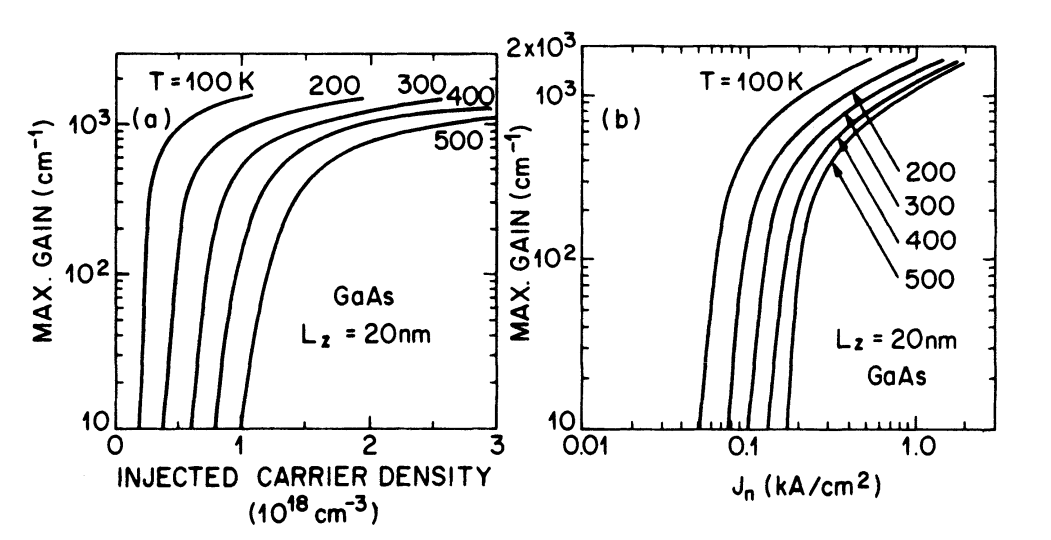Figure 9-8.  Calculated maximum gain for a GaAs quantum-well structure at various temperatures as a function of (a) the injected carrier density and (b) the nominal current density.

Figure 9-8 shows that the gain increases rapidly with the carrier density $$n$$ initially and then starts to saturate, leading to a slow increase. Near room temperature the crossover occurs when $$n\approx1\times10^{18}\text{ cm}^{-3}$$. The physical reason behind the gain saturation is related to the constant density of states of the lowest subband of the quantum well.

We now calculate the threshold current density and compare the calculated value with the measured results.

The threshold gain $$g_\text{th}$$ of a laser is given by [see Equations (2-3-9) and (2-3-10) in the threshold condition and longitudinal modes of semiconductor lasers tutorial]

$\tag{9-4-13}\Gamma{g}_\text{th}=\alpha_\text{int}+\frac{1}{L}\ln\left(\frac{1}{R}\right)$

The calculated confinement factor $$\Gamma$$ for a 25-nm-thick GaAs-Al0..52Ga0.48As quantum well is 0.04. When $$\alpha_\text{int}=10\text{ cm}^{-1}$$, $$R=0.3$$, and $$L=380$$ μm, we find from Equation (9-4-13) that $$g_\text{th}\approx1,000\text{ cm}^{-1}$$.

Thus the threshold gain for single quantum-well lasers is an order of magnitude larger than that for regular double-heterostructure lasers. This difference is principally due to a smaller confinement factor for a single quantum well.

For the calculated $$g_\text{th}$$ of $$1,000\text{ cm}^{-1}$$, the estimated nominal current density $$J_\text{n}$$ using Figure 9-8 is $$550\text{ A/cm}^2$$. This compares well with the reported threshold current density of $$810\text{ A/cm}^2$$ for a 20-nm-thick GaAs quantum-well laser.

Equation (9-4-13) shows that the small value of the confinement factor makes the threshold gain, and hence the threshold current density, of quantum-well lasers very sensitive to internal loss, which are influenced by defects, nonradiative regions, and unpumped active regions in an injection laser.

It is generally observed that the threshold current of GaAs quantum-well lasers does not increase as rapidly with increasing temperature as does that for regular double heterostructure lasers ($$T_0\approx250-400\text{K}$$ when $$T\gt270\text{ K}$$ for quantum-well lasers, while $$T_0\approx200\text{ K}$$ for regular double-heterostructure lasers).

Figure 9-9 shows the calculated threshold current as a function of temperature for GaAs-Al0.52Ga0.48As quantum wells of different active-region thicknesses.

The calculated $$T_0$$ value from Figure 9-9 is $$\sim300\text{ K}$$ when $$300\text{ K}\lt{T}\lt400\text{ K}$$. The reason for the high $$T_0$$ value is a weaker dependence of quasi-Fermi energies on temperature in this essentially two-dimensional system than in bulk semiconductors.

Since the condition for gain (at energy $$E$$) is given by $$E_\text{fc}+E_\text{fv}\gt{E-E_\text{g}}$$, fewer additional carriers at threshold are needed at a higher temperature for a quantum-well laser than for a regular double-heterostructure laser.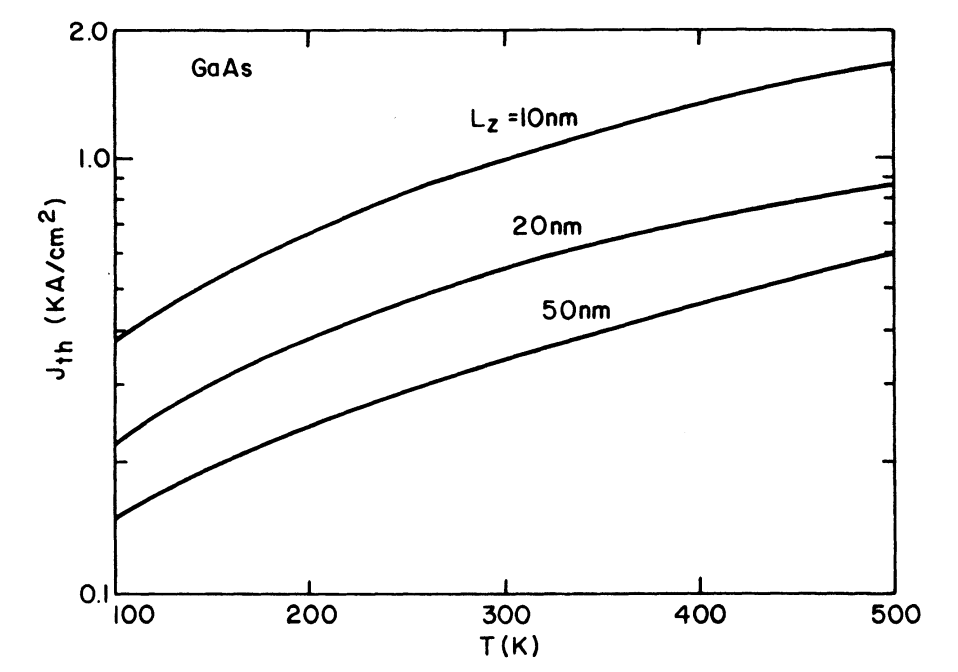Figure 9-9.  Calculated temperature dependence of the threshold current of a GaAs single quantum-well laser. Three curves for different well thicknesses are shown.

Figure 9-10 shows the calculated gain as functions of the injected carrier density and the nominal current density at various temperatures for a 1.3-μm InGaAsP-InP quantum-well structure. The parameter values used in the calculation are $$m_\text{c}=0.061m_0$$, $$m_\text{v}=0.45m_0$$, and $$E_\text{q}=1\text{ eV}$$.

Two curves for a 20-nm-thick well are shown at 300 K and 400 K. Similar to the case of GaAs (Figure 9-8), the gain saturates at high carrier densities because of the constant density of states of the lowest subband.Figure 9-10.  Calculated maximum gain for a 1.3-μm InGaAsP-InP quantum-well laser with a well thickness $$L_z$$ of 20 nm, as a function of (a) the injected carrier density and (b) the nominal current density. Gain variation at two different temperatures is shown.

Sugimura has calculated the radiative recombination rate in quantum-well structures by taking into account transitions between all subbands (in the $$\Gamma$$ valley) as well as the effect of filling the $$L$$ valleys.

Figure 9-11 shows the dependence of the gain on the well thickness $$L_z$$ for different injected carrier densities.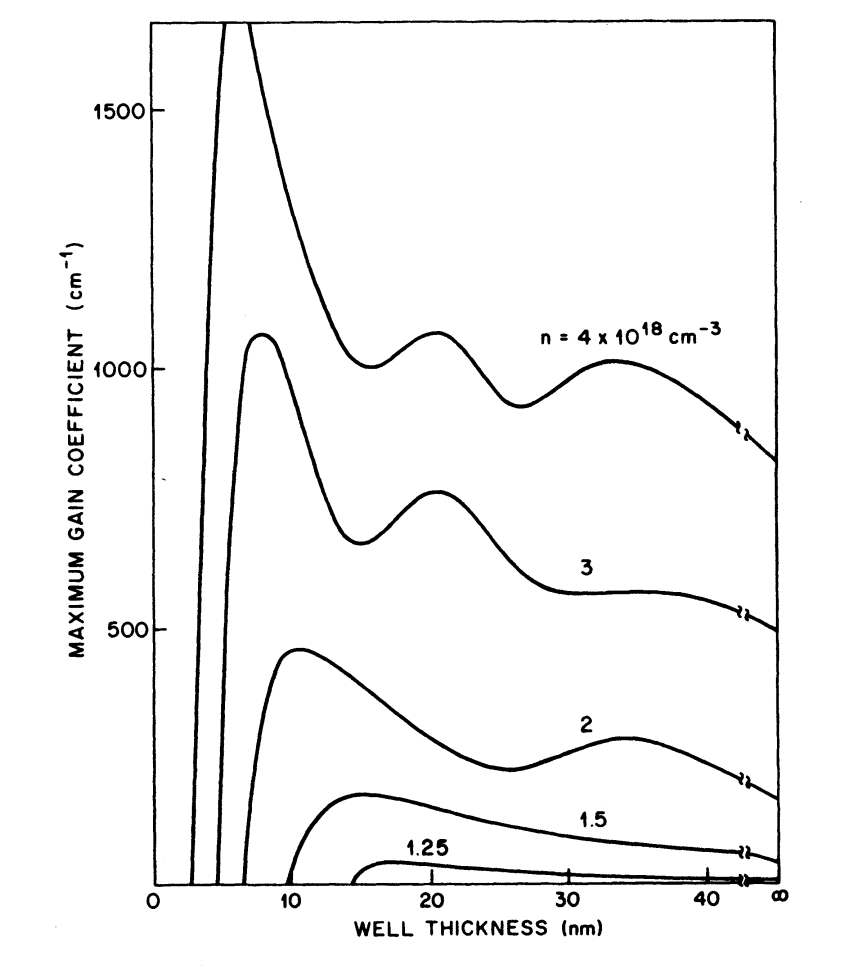Figure 9-11.  Maximum gain for an undoped InGaAsP-InP ($$\lambda\approx1.07$$ μm) single quantum-well laser as a function of well thickness for several values of injected carrier density.

The undulations in the value of the gain arise from recombinations between electrons in different subbands of the conduction band and the heavy or light holes in the valence band.

The steep decrease in the gain coefficient for small well thicknesses arises from the distribution of carriers in the $$L$$ valley, which reduces the number of carriers in the $$\Gamma$$ valley that contribute to optical gain.

Holonyak and coworkers have postulated the occurrence of phonon-assisted stimulated emission in quantum-well structures in order to explain their emission spectrum. However, the calculated phonon-assisted gain obtained using LO (longitudinal optical) phonon interactions is smaller than the gain obtained for direct transitions.

## 5. Auger Recombination

Auger recombination is an important nonradiative recombination mechanism for low band-gap materials. In the nonradiative recombination in semiconductors tutorial we discussed the importance of Auger recombination in determining the threshold current of long-wavelength InGaAsP lasers with relatively thick active regions.

The observed high temperature sensitivity of the threshold current of long-wavelength regular double-heterostructure lasers is generally attributed to Auger recombination. In quantum-well structures, the modification of the density of states may reduce the Auger recombination rate significantly to allow long-wavelength quantum-well lasers to have a relatively low temperature sensitivity.

We now outline the Auger-rate calculations for quantum-well structures.

Figure 9-12 shows the various band-to-band Auger processes. These processes are labeled CCCH, CHHS, and CHHL in analogy with the corresponding processes in bulk semiconductors [refer to the nonradiative recombination in semiconductors tutorial].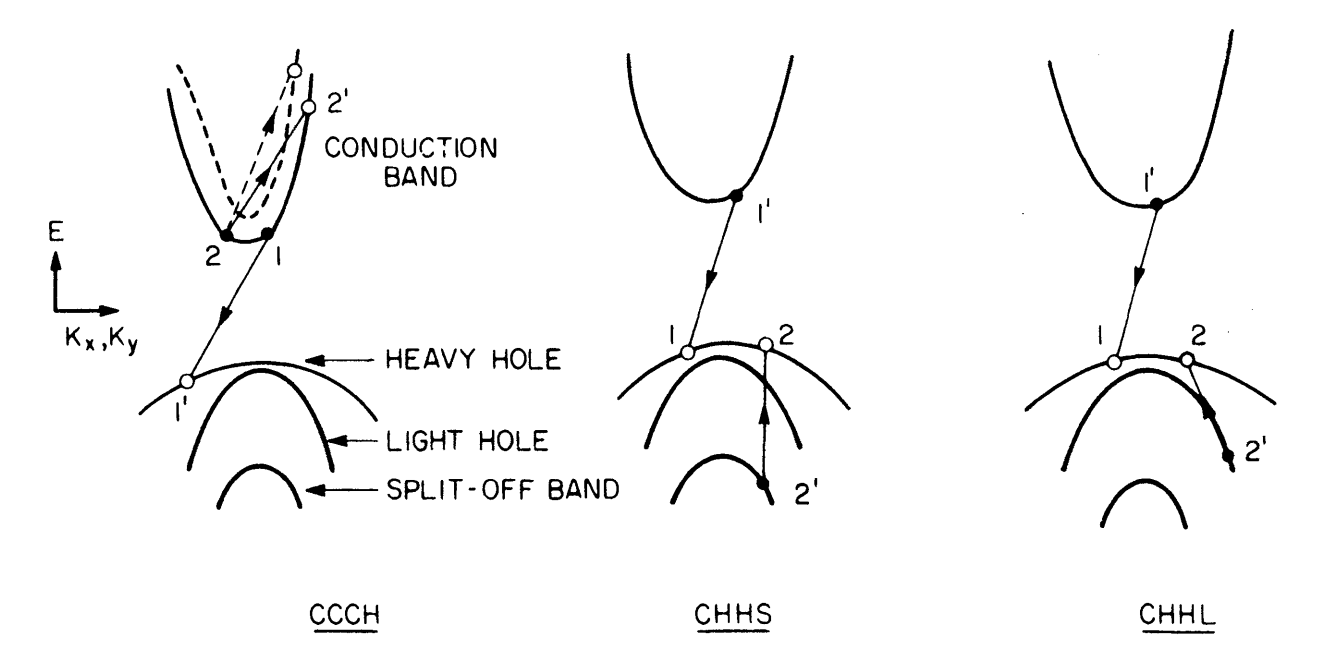Figure 9-12.  Band-to-band Auger recombination processes shown schematically for a quantum-well laser. Dashed curve shows the possibility of inter-subband scattering.

The energy-versus-wavevector diagram is shown for one subband (one state in the $$z$$ direction) in each of the bands. The dashed curve (for CCCH) shows a second subband in the conduction band. The dashed line shows a process in which the electron makes a transition to a different subband.

CCCH involves two electrons and a hole and is dominant in $$n$$-type material; CHHS and CHHL are dominant in $$p$$-type material. Under high-injection conditions, which are present in semiconductor lasers, all three mechanisms must be considered.

In this tutorial we derive expressions for the transition probability of these band-to-band Auger processes.

CCCH Process

In the nonradiative recombination in semiconductors tutorial we derived an expression for the CCCH Auger rate for bulk semiconductors [refer to Equation (3-3-3) in the nonradiative recombination in semiconductors tutorial].

The corresponding expression for the quantum-well structure is

\tag{9-5-1}\begin{align}R_\text{a}=&\frac{2\pi}{\hbar}\left(\frac{1}{(2\pi)^2}\right)^4\frac{1}{L_z}\\&\times\displaystyle\iiiint|M_\text{if}|^2P(1,1',2,2')\delta(E_\text{i}-E_\text{f})\text{d}^2\mathbf{k}_1\text{d}^2\mathbf{k}_2\text{d}^2\mathbf{k}_1'\text{d}^2\mathbf{k}_2'\end{align}

where $$R_\text{a}$$ denotes the Auger rate per unit volume. The quantity $$|M_\text{if}|^2$$ denotes the square of the matrix element and $$P(1,1',2,2')$$ is the difference between the probability of the Auger process shown in Figure 9-12 and the probability of the inverse process of impact ionization. The factor $$1/L_z$$ arises from converting the Auger rate per unit area (in two dimensions) to a per unit volume.

Equation (9-5-1) is a conversion of Equation (3-3-3) [refer to the nonradiative recombination in semiconductors tutorial] to two dimensions.

Using the same approximation used in the nonradiative recombination in semiconductors tutorial, we get $$|M_\text{if}|^2\approx4|\overline{M}_\text{if}|^2$$.

The quantity $$\overline{M}_\text{if}$$ is the matrix element for Coulomb interaction between two electrons and is given by

$\tag{9-5-2}\overline{M}_\text{if}=\displaystyle\iint\psi_1'^*(\mathbf{r}_1)\psi_2'^*(\mathbf{r}_2)\frac{q^2}{\epsilon|\mathbf{r}_1-\mathbf{r}_2|}\psi_1(\mathbf{r}_1)\psi_2(\mathbf{r}_2)\text{d}^3\mathbf{r}_1\text{d}^3\mathbf{r}_2$

where $$\psi_m(\mathbf{r})$$ are the quantum-well wave functions. Free carrier screening has been neglected in Equation (9-5-2).

To evaluate $$\overline{M}_\text{if}$$, we use the Fourier transform of the Coulomb interaction:

$\tag{9-5-3}\frac{1}{|\mathbf{r}_1-\mathbf{r}_2|}=\frac{1}{(2\pi)^3}\displaystyle\int\frac{4\pi}{\boldsymbol{\xi}^2}\exp[\text{i}\boldsymbol{\xi}\cdot(\mathbf{r}_1-\mathbf{r}_2)]\text{d}^3\boldsymbol{\xi}$

and obtain

$\tag{9-5-4}\overline{M}_\text{if}=\frac{4\pi{q^2}}{\epsilon}\frac{1}{(2\pi)^3}\displaystyle\int\frac{1}{\boldsymbol{\xi}^2}I_{1'1}(\boldsymbol{\xi})I_{2'2}(-\boldsymbol{\xi})\text{d}^3\boldsymbol{\xi}$

where

$\tag{9-5-5}I_{mn}(\boldsymbol{\xi})=\displaystyle\int\psi_m^*(\mathbf{r})\psi_n(\mathbf{r})\exp(\text{i}\boldsymbol{\xi}\cdot\boldsymbol{\rho})\text{d}^3\boldsymbol{r}$

The particle states in a quantum well are represented by the product of a localized Bloch function, a plane-wave term, and a confined-state wave function for the $$z$$ direction; i.e.,

$\tag{9-5-6}\psi_m(\mathbf{r})=U_m(\mathbf{r})\phi_m(z)\exp(-\text{i}\mathbf{k}_m\cdot\boldsymbol{\rho})$

where $$U_m(\mathbf{r})$$ is the localized part, $$\phi_m(z)$$ is the confined-state wave function, $$\mathbf{k}_m$$ is the two-dimensional wave vector in the plane of the well, and $$\boldsymbol{\rho}=(x,y)$$ is the two-dimensional position vector.

For an infinite well we can use Equation (9-1-3b) for $$\psi_n$$ in Equation (9-5-5) and evaluate $$I_{mn}(\boldsymbol{\xi})$$. The result is

$\tag{9-5-7}I_{mn}(\boldsymbol{\xi})=(2\pi)^2\delta(-\mathbf{k}_m+\mathbf{k}_n+\boldsymbol{\xi}_\text{T})F_{mn}G_{mn}(\xi_z)$

where

\begin{align}\boldsymbol{\xi}&=\boldsymbol{\xi}_\text{T}+\xi_z\mathbf{z}\\F_{mn}&=\displaystyle\int{U}_m^*(\mathbf{r})U_n(\mathbf{r})\text{d}^3\mathbf{r}\\G_{mn}&=\displaystyle\int\psi_m^*(z)\psi_n(z)\exp(\text{i}\xi_zz)\text{d}z\end{align}

$$\boldsymbol{\xi}_\text{T}$$ is the component of $$\boldsymbol{\xi}$$ parallel to the well and the integral $$F_{mn}$$ is carried out over a unit cell.

The matrix element $$\overline{M}_\text{if}$$ is now given by

$\tag{9-5-8}\overline{M}_\text{if}=\frac{2\pi{q}^2}{\epsilon}V\delta(\mathbf{k}_1+\mathbf{k}_2-\mathbf{k}_1'-\mathbf{k}_2')$

where

$\tag{9-5-9}V=4\pi{F_{11'}}F_{22'}\displaystyle\int\frac{G_{11'}G_{22'}}{|\mathbf{k}_1-\mathbf{k}_1'|^2+\boldsymbol{\xi}_z^2}\text{d}\xi_z$

In deriving the above, we have used the relation

$\boldsymbol{\xi}^2=\boldsymbol{\xi}_\text{T}^2+\xi_z^2=|\mathbf{k}_1-\mathbf{k}_1'|^2+\xi_z^2$

Using Equations (9-5-1) and (9-5-8), the Auger rate is given by

$\tag{9-5-10}R_\text{a}=\frac{8\pi}{\hbar}\left(\frac{1}{4\pi^2}\right)^4\frac{1}{L_z}\left(\frac{2\pi{q^2}}{\epsilon}\right)^2I$

where

\tag{9-5-11}\begin{align}I=&\displaystyle\iiiint{P}(1,1',2,2')\text{d}^2\mathbf{k}_1\text{d}^2\mathbf{k}_1'\text{d}^2\mathbf{k}_2\text{d}^2\mathbf{k}_2'|V(\mathbf{k}_1,\mathbf{k}_1')|^2\\&\times\delta(\mathbf{k}_1+\mathbf{k}_2-\mathbf{k}_1'-\mathbf{k}_2')\delta(E_\text{i}-E_\text{f})\end{align}

From the requirement of conservation of energy, it follows that

$\frac{\hbar^2}{2m_\text{c}}k_1^2+\frac{\hbar^2}{2m_\text{c}}k_2^2=\frac{\hbar^2}{2m_\text{v}}k_1'^2-E_\text{q}+\frac{\hbar^2}{2m_\text{ct}}k_2'^2$

where $$m_\text{c}$$ and $$m_\text{v}$$ are the electron and hole effective masses at the band edge and $$m_\text{ct}$$ is the electron effective mass at energy $$E_2'$$.

We now evaluate the integral $$I$$ by following the procedure of the nonradiative recombination in semiconductors tutorial.

Defining the new integration variables

$\mathbf{h}=\mathbf{k}_1+\mathbf{k}_2\qquad\qquad\mathbf{j}=\mathbf{k}_1-\mathbf{k}_2$

and integrating over them, we obtain

$\tag{9-5-12}I=\frac{m_\text{c}}{2\hbar^2}\displaystyle\iint\text{d}^2\mathbf{k}_1'\text{d}^2\mathbf{k}_2'\displaystyle\int\limits_{-1}^1\text{d}tP(1,1',2,2')|V(\mathbf{k}_1,\mathbf{k}_1')|^2|\mathbf{j}_0|$

where $$t=\cos\theta$$ and $$\theta$$ is the angle between the vectors $$\mathbf{h}$$ and $$\mathbf{j}$$.

The integral is to be evaluated under the condition $$j_0^2\ge0$$, where

\begin{align}j_0^2&=2\left(\mu_\text{c}k_2'^2-\mu{k}_1'^2-\frac{2m_\text{c}E_\text{q}}{\hbar^2}\right)-|\mathbf{k}_1'+\mathbf{k}_2'|^2\\\mu_\text{c}&=\frac{m_\text{c}}{m_\text{ct}}\qquad\mu=\frac{m_\text{c}}{m_\text{v}}\end{align}

If we define two new variables

$\tag{9-5-13}\mathbf{z}_1=\mathbf{k}_1'+\frac{\mathbf{k}_2'}{1+2\mu}\qquad\mathbf{z}_2=\mathbf{k}_2'$

$$j_0^2$$ becomes

$\tag{9-5-14}j_0^2=2\alpha_\text{c}z_2^2-z_1^2(1+2\mu)-\frac{4m_\text{c}}{\hbar^2}E_\text{q}$

where

$\tag{9-5-15}\alpha_\text{c}=\mu_\text{c}-\frac{\mu}{1+2\mu}$

A necessary condition for $$j_0^2\ge0$$ is

$\alpha_\text{c}z_2^2\gt\frac{2m_\text{c}E_\text{q}}{\hbar^2}$

or, using $$E_2'=\hbar^2z_2^2/2m_\text{c}$$,

$\tag{9-5-16}E_2'\gt{E}_\text{T}=\mu_\text{c}E_\text{q}/\alpha_\text{c}$

Thus, similar to the three-dimensional case $$E_2'$$ must reach a threshold energy ($$E_\text{T}=\mu_\text{c}E_\text{q}/\alpha_\text{c}$$) before the Auger process will take place.

The quantity $$P(1,1',2,2')$$ is the difference between the product of the occupation probabilities of the four states for the Auger process and those for the inverse process of impact ionization. When $$E_\text{q}\gg{k}_\text{B}T$$, the inverse process is relatively unlikely to occur and can be ignored.

If we further make the nondegenerate approximation [Equation (9-2-9)], $$P(1,1',2,2')$$ is given by

$\tag{9-5-17}P(1,1',2,2')=\frac{n^2p}{N_\text{c}^2N_\text{v}}\exp\left(\frac{-E_2'+E_\text{q}}{k_\text{B}T}\right)$

where $$n$$ and $$p$$ are the electron and hole concentrations, respectively, and we have used the energy conservation relation

$\tag{9-5-18}E_1+E_2=E_2'-E_\text{q}-E_1'$

Since the exponents in Equation (9-5-17) decreases rapidly as $$E_2'$$ increasses, we can approximate $$|V|^2$$ in Equation (9-5-12) by its value at $$E_2'=E_\text{T}$$. Using Equations (9-5-13) and (9-5-17) in Equation (9-5-12), we finally obtain

$\tag{9-5-19}I=\frac{m_\text{c}}{\hbar^2}\frac{n^2p}{N_\text{c}^2N_\text{v}}|V_\text{T}|^2\exp\left(\frac{E_\text{q}}{k_\text{B}T}\right)I_1$

where

\tag{9-5-20}\begin{align}I_1&=\displaystyle\iint\text{d}^2z_1\text{d}^2z_2\exp\left(-\frac{\hbar^2z_2^2}{2m_\text{ct}k_\text{B}T}\right)\\&=\frac{8\pi^2\alpha_\text{c}}{1+2\mu}\left(\frac{2m_\text{ct}k_\text{B}T}{\hbar^2}\right)^2\exp\left(-\frac{m_\text{c}}{m_\text{ct}\alpha_\text{c}}\frac{E_\text{q}}{k_\text{B}T}\right)\end{align}

and $$|V_\text{T}|^2$$ is the value of $$|V|^2$$ at $$E_2'=E_\text{T}$$.

The integral $$I_1$$ has been evaluated under the condition $$j_0^2\ge0$$, where $$j_0$$ is given by Equation (9-5-14) and $$m_\text{ct}$$ is the electron effective mass at the energy $$E_\text{T}$$.

Using Equations (9-5-10) and (9-5-19) we get in the nondegenerate approximation

$\tag{9-5-21}R_\text{a}\propto\frac{n^2p}{N_\text{c}^2N_\text{v}}\exp\left(-\frac{\Delta{E}_\text{c}}{k_\text{B}T}\right)$

where

$\tag{9-5-22}\Delta{E}_\text{c}=E_\text{T}-E_\text{q}=\frac{m_\text{ct}E_\text{q}}{m_\text{v}+2m_\text{c}-m_\text{ct}}$

Equations (9-5-21) and (9-5-22) show that the Auger rate increases with decreasing band gap and with increasing temperature.

CHHS Process

The CHHS Auger rate can be calculated using the same procedure as for CCCH.

The expression for the Auger rate per unit volume is still given by Equation (9-5-10). However, because of different particle states participating in the process, the integral $$I$$ given by Equation (9-5-11) is different.

Following the analysis of the nonradiative recombination in semiconductors tutorial for the CHHS process in bulk semiconductors, it can be shown that the threshold energy for the process is given by [refer to Equation (3-3-37) in the nonradiative recombination in semiconductors tutorial]

$\tag{9-5-23}E_\text{Ts}=\frac{\hbar^2k_\text{2t}'^2}{2m_\text{s}}=\frac{2m_\text{v}+m_\text{c}}{2m_\text{v}+m_\text{c}-m_\text{s}}(E_\text{q}-\Delta_1)$

where $$m_\text{s}$$ is the mass of the split-off band at energy $$E_\text{Ts}$$ and $$\Delta_1$$ is the energy separation of the split-off band from the top of the heavy-hole band.

This minimum energy requirement arises from the conservation laws of energy and momentum that the four particle states involved must satisfy.

In the nondegenerate approximation, the integral $$I$$ can be evaluated in a closed form after using Equation (9-5-17) in Equation (9-5-11) and is given by

$\tag{9-5-24}I=\frac{m_\text{c}}{\hbar^2}\frac{np^2}{N_\text{c}N_\text{v}^2}|V_\text{T}|^2\exp\left(\frac{E_\text{q}-\Delta_1}{k_\text{B}T}\right)I_1$

where

$\tag{9-5-25}I_1=\frac{8\pi^2\alpha_\text{s}}{(1+\mu/2)}\left(\frac{2m_\text{s}k_\text{B}T}{\hbar^2}\right)^2\exp\left[-\frac{m_\text{c}}{\alpha_\text{s}m_\text{s}}(E_\text{q}-\Delta_1)\right]$

$\tag{9-5-26}\alpha_\text{s}=\mu_\text{s}-1+\frac{1}{1+\mu/2}$

$\tag{9-5-27}\mu_\text{s}=m_\text{c}/m_\text{s}\qquad\mu=m_\text{c}/m_\text{v}$

Using Equations (9-5-10) and (9-5-24), the Auger rate is found to vary as (in the nondegenerate approximation)

$\tag{9-5-28}R_\text{a}\propto{p^2}n\exp\left(-\frac{\Delta{E}_\text{s}}{k_\text{B}T}\right)$

where

$\tag{9-5-29}\Delta{E}_\text{s}=E_\text{Ts}-E_\text{q}=\frac{m_\text{s}(E_\text{q}-\Delta_1)}{m_\text{c}+2m_\text{v}-m_\text{s}}$

This formulation is valid for semiconductors where $$E_\text{q}\gt\Delta_1$$. Similar to the case of CCCH, the CHHS Auger rate increases with increasing temperature and as the term $$E_\text{q}-\Delta_1$$ decreases.

CHHL Process

The calculation for this process proceeds in an identical fashion as for CHHS. The results given there can be directly applied by setting $$\Delta_1$$ to $$0$$ and replacing $$m_\text{s}$$ by $$m_\text{l}$$ where $$m_\text{l}$$ is the light-hole mass.

The Auger recombination rate per unit volume is given by Equation (9-5-10), while the threshold energy for the process is [refer to Equation (9-5-23)]

$\tag{9-5-30}E_\text{Tl}=\frac{\hbar^2k_\text{2t}'^2}{2m_\text{l}}=\frac{2m_\text{v}+m_\text{c}}{2m_\text{v}+m_\text{c}-m_\text{l}}E_\text{q}$

In the nondegenerate approximation $$I$$ is given by Equations (9-5-24) and (9-5-25) with $$m_\text{s}$$, $$\alpha_\text{s}$$, and $$E_\text{q}-\Delta_1$$ replaced by $$m_\text{l}$$, $$\alpha_\text{l}$$, and $$E_\text{q}$$, respectively. The Auger rate varies as

$\tag{9-5-31}R_\text{a}\propto{p^2}n\exp\left(-\frac{\Delta{E}_\text{l}}{k_\text{B}T}\right)$

where

$\tag{9-5-32}\Delta{E}_\text{l}=\frac{m_\text{l}E_\text{q}}{m_\text{c}+2m_\text{v}-m_\text{l}}$

The expressions for the three Auger processes derived here show that the Auger rate increases with decreasing band gap and with increasing temperature. They can be used to estimate the importance of Auger recombination for quantum-well lasers by calculating the Auger lifetime $$\tau_\text{A}=n/R_\text{a}$$.

For CCCH the calculated Auger lifetime depends strongly on the value of $$\Delta{E}_\text{c}$$ given by Equation (9-5-22).

For bulk semiconductors, $$m_\text{ct}$$ (the conduction band mass at the threshold energy $$E_\text{T}$$) is obtained using the Kane model, which gives $$m_\text{ct}\approx1.7m_\text{c}$$.

For a quantum-well laser, the ratio $$m_\text{ct}/m_\text{c}$$ is not known.

For parabolic bands, $$m_\text{ct}/m_\text{c}=1$$ and Equation (9-5-22) reduces to

$\tag{9-5-33}\Delta{E}_\text{c}=\mu{E_\text{q}}/(1+\mu)$

where $$\mu=m_\text{c}/m_\text{v}$$.

The calculated Auger rate for a given band gap can decrease by more than one order of magnitude if the ratio $$m_\text{ct}/m_\text{c}$$ increases from 1 to 2.

Under high-injection conditions commonly prevailing in semiconductor lasers, it is often necessary to go beyond the nondegenerate approximation. The Auger rate is then calculated numerically using Equations (9-5-10) and (9-5-11).

Figure 9-13 shows the calculated Auger lifetime $$\tau_\text{A}$$ for a 1.07-μm InGaAsP quantum-well laser as a function of the well thickness $$L_z$$ for several carrier densities $$n$$. The active region is assumed to be undoped.Figure 9-13.  Calculated Auger lifetime for CHHS in an undoped InGaAsP-InP quantum-well laser as a function of the well thickness for several values of the injected carrier density.

The undulations in the vicinity of $$L_z\sim$$ 10 nm are due to the filling of the higher-order subbands with increasing $$n$$.

One can estimate the value of the Auger coefficient $$C$$ that is defined using

$\tag{9-5-34}R_\text{a}=n/\tau_\text{A}=Cn^3$

If $$L_z=30$$ nm, $$n=2\times10^{18}\text{ cm}^{-3}$$ (typical values), $$C\approx2.5\times10^{-29}\text{ cm}^6/\text{s}$$ from Figure 9-13.

However, it should be stressed that because of the uncertainties of both the matrix elements and the effective masses away from the band edge (the ratio $$m_\text{ct}/m_\text{c}$$), only order-of-magnitude estimates of the Auger rates can be obtained.

## 6. Single Quantum-Well and Multiquantum-Well Lasers

Quantum-well injection lasers with both single and multiple active layers have been fabricated. Quantum-well lasers with one active region are called single quantum-well (SQW) lasers, and those with multiple active regions are called multiquantum-well (MQW) lasers.

The layers separating the active layers in an MQW structure are called barrier layers. Figure 9-14 schematically shows the energy-band diagram of these laser structures. The MQW lasers in which the band gap of the barrier layers is different from that of the cladding layers are sometimes referred to as modified multiquantum-well lasers.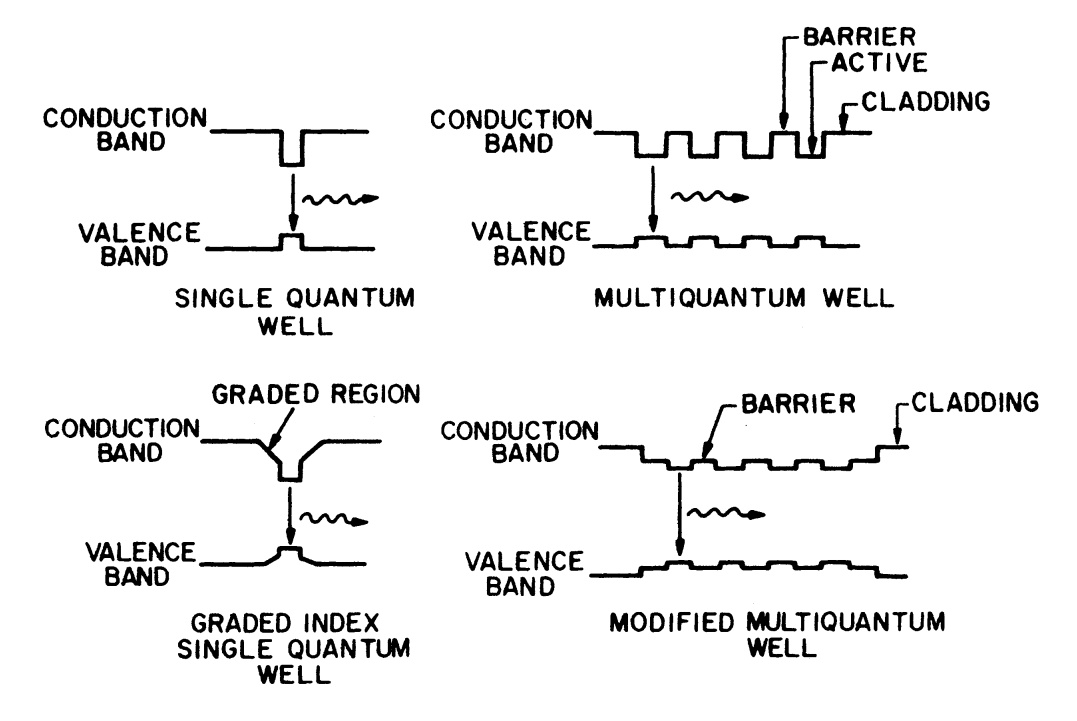Figure 9-14.  Different single quantum-well and multiquantum-well laser structures shown schematically.

One of the main differences between the SQW and MQW lasers is that the confinement factor $$\Gamma$$ of the optical mode is significantly smaller for the former than that for the latter. This can result in a higher threshold carrier density and a higher threshold current density for SQW lasers when compared with MQW lasers.

The confinement factor of an SQW heterostructure can be significantly increased using a graded-index (GRIN) cladding layer (see Figure 9-14). This allows use of the intrinsic advantage of the quantum-well structure (high gain at low carrier density) without the penalty of a small mode-confinement factor. Threshold current densities as low as $$200\text{ A/cm}^2$$ have been reported for a GaAs GRIN SQW laser.

We now discuss the mode-confinement factor in SQW and MQW structures.

The confinement factor of the fundamental mode of a double heterostructure was calculated in the waveguide modes in semiconductor lasers tutorial and is given approximately by Equation (2-5-32).

For a small active-layer thickness, such as that of a single quantum well, the expression for $$\Gamma$$ can be further simplified since the normalized waveguide thickness $$D\ll1$$. Using Equations (2-5-26) and (2-5-32) [refer to the waveguide modes in semiconductor lasers tutorial], we then obtain

$\tag{9-6-1}\Gamma\approx2\pi^2(\mu_\text{a}^2-\mu_\text{c}^2)d^2/\lambda_0^2$

where $$\mu_\text{a}$$ and $$\mu_\text{c}$$ are the refractive indices of the active and cladding layers, respectively; $$d$$ is the active-layer thickness; and $$\lambda_0$$ is the free-space wavelength.

Figure 9-15 shows the calculated $$\Gamma$$ obtained using Equation (9-6-1) for InGaAsP-InP SQW structures emitting at different wavelengths.Figure 9-15.  Calculated confinement factor of the fundamental mode as a function of the active-layer thickness for an InGaAsP-InP single quantum-well heterostructure. The refractive indices of the active and cladding layers used in the calculations are: (i) $$\lambda$$ = 1.07 μm, $$\mu_\text{a}$$ = 3.47, $$\mu_\text{c}$$ = 3.27; (ii) $$\lambda$$ = 1.3 μm, $$\mu_\text{a}$$ = 3.51, $$\mu_\text{c}$$ = 3.22; (iii) $$\lambda$$ = 1.55 μm, $$\mu_\text{a}$$ = 3.54, $$\mu_\text{c}$$ = 3.18.

The mode confinement in MQW structures can be analyzed using the same procedure as described in the waveguide modes in semiconductor lasers tutorial, except that the electromagnetic wave equation must be solved separately, with appropriate boundary conditions, for each of the active, barrier, and cladding layers.

This procedure is quite tedious because of a large number of layers involved. The following simple formula is found to give reasonably accurate results:

$\tag{9-6-2}\Gamma(\text{MQW})=\gamma\frac{N_\text{a}d_\text{a}}{N_\text{a}d_\text{a}+N_\text{b}d_\text{b}}$

where

$\tag{9-6-3}\gamma=2\pi^2(N_\text{a}d_\text{a}+N_\text{b}d_\text{b})^2\frac{\bar{\mu}^2-\mu_\text{c}^2}{\lambda_0^2}$

$\tag{9-6-4}\bar{\mu}=\frac{N_\text{a}d_\text{a}\mu_\text{a}+N_\text{b}d_\text{b}\mu_\text{b}}{N_\text{a}d_\text{a}+N_\text{b}d_\text{b}}$

$$N_\text{a}$$ and $$N_\text{b}$$ are the number of active and barrier layers in the MQW structure, and $$d_\text{a}$$ and $$d_\text{b}$$ (and $$\mu_\text{a}$$ and $$\mu_\text{b}$$) are the thicknesses (and refractive indices) of the active and barrier layers, respectively.

The form of Equation (9-6-2) may be understood as follows: $$\bar{\mu}$$ is the average refractive index of an active region (consisting of all the active and barrier layers) of total thickness $$N_\text{a}d_\text{a}+N_\text{b}d_\text{b}$$, and $$\gamma$$ is the confinement factor of the optical mode [same as in Equation (9-6-1)] in this effective layer of index $$\bar{\mu}$$. The MQW mode-confinement factor $$\Gamma$$ is thus obtained by multiplying $$\gamma$$ by the ratio of the total active thickness $$N_\text{a}d_\text{a}$$ to the total thickness $$N_\text{a}d_\text{a}+N_\text{b}d_\text{b}$$.

For a 1.3-μm InGaAsP-InP multiquantum-well structure with four active-layer wells (each 15 nm thick) and three barrier layers (InP, each 15 nm thick), the calculated $$\Gamma\approx0.2$$. This is considerably larger than the typical $$\Gamma$$ values for SQW lasers (shown in Figure 9-15) with active-layer thicknesses in the range of 10-15 nm.

Let us now compare single quantum-well and multiquantum-well lasers for the effect of Auger recombination on the threshold current.

The gain at threshold ($$g_\text{th}$$) is given by Equation (2-3-9) [refer to the threshold condition and longitudinal modes of semiconductor lasers tutorial], which after using Equations (2-3-10) and (2-5-50) [refer to the waveguide modes in semiconductor lasers tutorial] becomes

$\tag{9-6-5}\Gamma{g_\text{th}}=\Gamma\alpha_\text{a}+(1-\Gamma)\alpha_\text{c}+\frac{1}{L}\ln\left(\frac{1}{R}\right)$

where $$\alpha_\text{a}$$ and $$\alpha_\text{c}$$ are the active and cladding layer losses, $$L$$ is the length of the laser, and $$R$$ is the reflectivity of the end mirrors.

For an SQW laser, the confinement factor $$\Gamma$$ is small and hence the threshold gain is very large ($$g_\text{th}\ge10^3\text{ cm}^{-1}$$). This makes the carrier density at threshold large: $$n_\text{th}\approx2.5\times10^{18}\text{ cm}^{-3}$$ (see Figure 9-10).

For an MQW laser, the effective confinement factor is large and $$g_\text{th}$$ is smaller ($$g_\text{th}\approx300\text{ cm}^{-1}$$). The carrier density at threshold $$n_\text{th}\approx8\times10^{17}\text{ cm}^{-3}$$ at this gain (see Figure 9-10).

The Auger rate varies as $$n^3$$. Thus it follows that the Auger rate in MQW laser will be significantly smaller (by a factor of 27 in our example) when compared with the Auger rate in SQW lasers.

Also for the same reason, any nonradiative mechanism that depends superlinearly on the carrier density will have a smaller effect in MQW lasers than in SQW lasers.

We next consider the effect of increased optical losses with increasing temperature (e.g., intervalence-band absorption).

For SQW lasers, $$\Gamma$$ is small and hence the first term on the right-hand side of Equation (9-6-5) is negligible. Thus the effect of increased intervalence-band absorption in determining the temperature dependence of threshold is smaller in SQW lasers than in MQW lasers.

Another point to note is that for $$g_\text{th}\ge10^3\text{ cm}^{-1}$$ (near the threshold-gain region of SQW lasers) the gain increases slowly with increasing current (see Figure 9-10). This will make the threshold current of SQW lasers more sensitive to increased cladding layer losses, which may increase with increasing temperature because free-carrier absorption is larger at higher temperatures.

## 7. MQW Laser Results

A considerable amount of experimental work has been reported on quantum-well lasers fabricated using the AlGaAs material. The epitaxial layers are grown using molecular beam epitaxy (MBE) or metal-organic chemical vapor deposition (MOVPE) [refer to the what is vapor phase epitaxy tutorial].

The performance of AlGaAs lasers grown by MBE technique has been reviewed. The results show that AlGaAs quantum-well lasers exhibit a lower threshold current, a somewhat higher differential quantum efficiency, and a weaker temperature dependence of threshold current when compared with regular double-heterostructure lasers.

Some of the results that demonstrate the high performance of AlGaAs quantum-well lasers are:

1. Fabrication of GRIN SQW lasers with the lowest reported threshold current density ($$200\text{ A/cm}^2$$, which compares with values in the range of $$0.6-1\text{ kA/cm}^2$$ for regular double-heterostructure lasers).
2. Fabrication of GRIN SQW buried-heterostructure lasers with the lowest reported threshold current (2.5 mA).
3. Fabrication of MQW lasers with the threshold current density of $$250\text{ A/cm}^2$$.
4. Fabrication of laser arrays with MQW active regions that have been operated to very high CW power (more than 2 W).

Also, some of the studies relating to the differences between the gain and recombination mechanisms in quantum-well structures and regular double heterostructures have been carried out using the AlGaAs material system. These studies include polarization dependence of gain, spectral characteristics of gain, and measurements of carrier lifetime.

Rezek and coworkers first reported on the growth of InGaAsP quantum-well structures by liquid-phase epitaxy (LPE). The room-temperature threshold current density of these lasers was $$\sim5.2\text{ kA/cm}^2$$. Figure 9-16 shows the measured temperature dependence of the threshold current of a MQW laser with 12 active layers of thickness ~ 16 nm each.Figure 9-16.  Measured temperature dependence of threshold current of an InGaAsP MQW laser. Solid and dashed lines show the exponential fit below and above the break-point temperature occurring near room temperature.

One of the interests in studying InGaAsP quantum-well structures is to examine the temperature dependence of the threshold current.

In the temperature dependence of threshold current of semiconductor lasers tutorial we showed that the threshold current density as a function of temperature may be written as $$J_\text{th}(T)\approx{J_0}\exp(T/T_0)$$.

For regular double-heterostructure lasers, $$T_0\approx110\text{ K}$$ when $$T\lt250\text{ K}$$ and $$T_0\approx60\text{ K}$$ when $$T\gt250\text{ K}$$. The temperature at which $$T_0$$ changes is often called the break-point temperature ($$T_\text{b}$$).

Figure 9-16 shows that $$T_\text{b}\approx300\text{ K}$$ for MQW lasers, which suggest that they have a somewhat lower temperature sensitivity compared with that of regular InGaAsP double-heterostructure lasers, but not as low as that of GaAs double-heterostructure lasers.

InGaAsP double-channel planar buried-heterostructure (DCPBH) lasers with a multiquantum-well active layer have also been fabricated. The MQW structure was grown by LPE. Two types of MQW structures were studied: (i) with InP barrier layers and (ii) with InGaAsP ($$\lambda\approx1.0\text{ μm}$$) barrier layers.

The MQW active region had four active wells ($$\lambda\approx1.3$$ μm, InGaAsP) and three barrier layers. Figure 9-17 shows a schematic cross section of the DCPBH laser and the MQW structure of its active layer.

DCPBH lasers with InP barrier layer had room temperature threshold currents in the range of 40-50 mA, while for DCPBH lasers with InGaAsP barrier layers ($$\lambda\approx1.03$$ μm), the threshold currents were in the range 20-25 mA. The lower threshold current of devices with a lower barrier height may be due to more uniform current injection. A similar observation was made for AlGaAs MQW lasers.Figure 9-17.  Schematic cross sections of the DCPBH laser and its MQW active region. Details of the active region are shown on the right for (a) InP and (b) 1.03-μm InGaAsP barrier layers.

Figure 9-18 shows the CW light-current characteristics at different temperatures of an InGaAsP DCPBH MQW laser and should be compared with those shown in Figure 5-25 [refer to the strongly index-guided semiconductor lasers tutorial] for regular double-heterostructure lasers.

In both cases the threshold current $$I_\text{th}\approx{I_0}\exp(T/T_0)$$. However, for MQW lasers the characteristic temperature ($$T_0\approx100\text{ K}$$) is higher than for the regular double-heterostructure lasers ($$T_0\approx60\text{ K}$$), suggesting that the former are less sensitive to temperature increases.Figure 9-18.  Light-current characteristics of an InGaAsP MQW laser at different temperatures.

InGaAsP-InP MQW lasers emitting near 1.3 μm have also been fabricated using the hydride-transport VPE technique. Figure 9-19 shows the threshold current density measured in those broad-area lasers as a function of well thickness.

Also shown in the figure are the measured $$T_0$$ values that are comparable tot the values reported for regular InGaAsP double-heterostructure lasers. The number and thickness of quantum wells used in these lasers were such that the total active thickness ($$\sim0.2$$ μm) was relatively constant for the three devices shown.Figure 9-19. Measured threshold current density $$J_\text{th}$$ (solid curve) and characteristic temperature $$T_0$$ (dashed curve) as a function of active-layer thickness for a 1.3- μm InGaAsP-InP MQW laser.

Quantum-well lasers emitting near 1.55 μm, the region of lowest optical loss in silica fibers, have also attracted considerable attention. Much of the work on InGaAsP/InP MQW lasers has been carried out using the MOVPE and CBE epitaxial growth techniques.

A separate-confinement heterostructure (SCH) is generally used to increase the confinement of the lasing mode to the active region. It has been shown that the optical loss of the mode can be reduced significantly, and hence high powers can be obtained, by a proper choice of he composition of the materials that form the GRIN region and the barrier layers.

The measured threshold current density for InGaAs MQW lasers grown by the MOVPE and CBE growth techniques is shown in Figure 9-20. The threshold current density is found to decrease with increasing cavity length with the lowest value being $$380\text{ A/cm}^2$$ for 2-mm cavity length.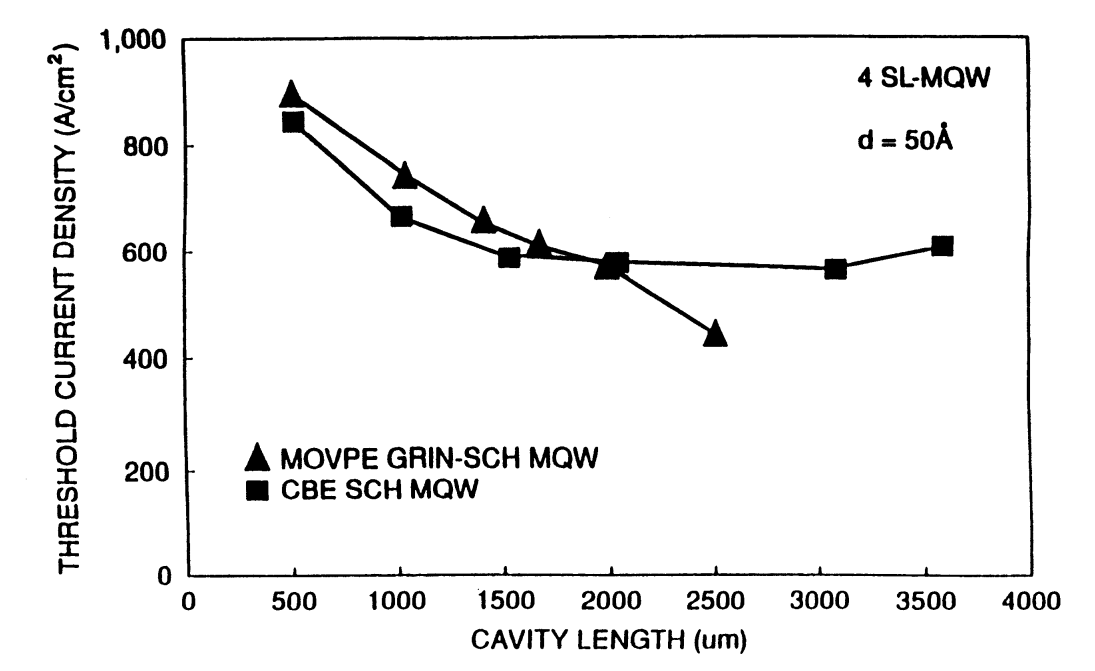Figure 9-20.  Threshold current density plotted as a function of cavity length for InGaAsP MQW lasers grown by the MOVPE and CBE techniques.

As discussed in the weakly index-guided lasers and strongly index-guided lasers tutorials, index guiding along the junction plane is necessary in order to control the near-field pattern of a semiconductor laser.

The schematic of an index-guided buried heterostructure laser is shown in Figure 9-21. The active-region width is ~ 2 μm. The laser utilizes Fe-doped InP layers for confinement of the current to the active region and for providing lateral index guiding.Figure 9-21.  Schematic illustration of an index-guided InGaAsP MQW laser. The band diagram (bottom) shows the graded-index region between the barrier and cladding regions.

Threshold current of a laser can be reduced by using high-reflectivity facet coatings. For QW lasers the reduction is larger than that for regular double-heterostructures because the transparency current density is lower for QW lasers. The lower transparency current density arises from the two-dimensional density of states in MQWs.

The optical gain for a laser with a current density $$J$$ is given by

$\tag{9-7-1}g=a(J-J_\text{tr})$

where $$a$$ is the gain constant, $$J_\text{tr}$$ is the transparency current density.

At threshold, gain equals total loss, and the latter is the sum of the free carrier and mirror losses, i.e.,

$\tag{9-7-2}g=a(J_\text{th}-J_\text{tr})=\alpha+\frac{1}{L}\ln\left(\frac{1}{R_1R_2}\right)$

where $$J_\text{th}$$ is the threshold current density, $$\alpha$$ is the free carrier loss, $$L$$ is the cavity length and $$R_1, R_2$$ are the mirror reflectivities.

It follows from Equation (9-7-2) that $$J_\text{th}$$ is given by

$\tag{9-7-3}J_\text{th}=J_\text{tr}+\frac{\alpha}{a}+\frac{1}{La}\ln\left(\frac{1}{R_1R_2}\right)$

By increasing $$R_1,R_2$$ and decreasing the cavity loss $$\alpha$$, $$J_\text{th}$$ can be made close to $$J_\text{tr}$$. Typically $$J_\text{tr}=50\text{ A/cm}^2$$ for QW lasers whereas for DH lasers it is $$\sim500\text{ A/cm}^2$$.

Threshold currents of both GaAs and InGaAsP MQW lasers have been reduced using high-reflectivity facet coatings. Such lasers are important for parallel data link applications.

InGaAsP MQW laser emitting near 1.55 μm with a threshold current of 1 mA have been realized. These lasers have 90% reflective coating at the back facet and 70% reflective coating at the front facet.

GaAs QW lasers with a threshold current of 0.35 mA have also been obtained. These lasers had 90% reflective coating on both facets.

## 8. Modulation and Noise Characteristics

For lightwave system applications, it is important to investigate if the quantum-well nature of a semiconductor laser affects other operating characteristics such as the relaxation-oscillation frequency, the laser line width, and the frequency chirp.

These characteristics were discussed in previous tutorials for regular double-heterostructure lasers. Among various parameters used there [refer to Table 6-1 in the rate equations for semiconductor lasers tutorial], the parameters that may be particularly influenced by quantum-size effects are the gain coefficient $$a$$, the carrier density at transparency $$n_0$$, and the line-width enhancement factor $$\beta_\text{c}$$.

Attempts have been made to analyze the dependence of these parameters on MQW design parameters such as the number and thickness of quantum wells.

The gain parameters $$a$$ and $$n_0$$ for regular double-heterostructure lasers are estimated using the linear-gain model $$g(n)=a(n-n_0)$$ described in the gain and stimulated emission in semiconductor lasers tutorial.

Although this model is reasonably accurate for those lasers [refer to Figure 3-8 in the radiative recombination in semiconductor lasers tutorial], the gain variation for quantum-well semiconductor lasers is far from linear (see Figures 9-8 and 9-10 above); the gain eventually saturates because of the constant density of states of the lowest subband [see Equation (9-2-3) above].

As a result, the gain coefficient defined as $$a=\text{d}g/\text{d}n$$ varies with the carrier density and would be different for different MQW lasers, depending on the structure parameters such as the number and thickness of quantum wells.

Calculations for SQW and MQW lasers show that with a proper design, the effective value of $$a$$ can be nearly doubled compared with that of regular semiconductor lasers. Equations (6-4-23) and (6-4-26) [refer to the transient response of semiconductor lasers tutorial] then show that, if other parameters are approximately the same, the relaxation-oscillation frequency $$\Omega_\text{R}$$ would increase by about 50%. An increase in $$\Omega_\text{R}$$ can enhance the modulation bandwidth [refer to the modulation response of semiconductor lasers tutorial] and decrease the intensity noise [refer to the noise characteristics of semiconductor lasers tutorial] at a given output power.

The line-width enhancement factor $$\beta_\text{c}$$ is defined by Equation (2-4-6) [refer to the gain and stimulated emission in semiconductor lasers tutorial]. This equation shows that if the carrier-induced index change is relatively unaffected, an increase in $$a$$ would lead to a decrease in $$\beta_\text{c}$$ by the same factor. A lower value of $$\beta_\text{c}$$ for quantum-well lasers has been predicted theoretically.

As discussed in previous tutorials, the main effect of this lower value would be to reduce the CW line width [see Equation (6-5-52) in the noise characteristics of semiconductor lasers tutorial] as well as the frequency chirp [see Equation (6-6-45) in the modulation response of semiconductor lasers tutorial].

The measurements of the frequency chirp for quantum-well semiconductor lasers shown in Figure 9-22 suggest that it is smaller by about a factor of 2 compared with the values obtained for regular double-heterostructure lasers. By the same token, the CW line width should reduce by about a factor of 4.

These results suggest that properly optimized MQW semiconductor lasers may be useful for applications in optical communication systems.Figure 9-22.  Wavelength chirp as a function of peak-to-peak modulation current for an MQW laser (solid line) and a regular double-heterostructure laser (dashed line).

## 9. Strained Quantum-Well Lasers

Quantum-well lasers have also been fabricated using an active layer whose lattice constant differs slightly from that of the substrate and cladding layers. Such lasers are known as strained quantum-well lasers.

Strained quantum-well lasers show many desirable properties such as (i) a very low threshold current density and (ii) a lower line width both under CW operation and under modulation.

The origin of the improved device performance lies in the band-structure changes induced by the mismatch-induced strain. Figure 9-23 shows the band structure of a semiconductor under tensile and compressive strains.

Strain splits the heavy-hole and the light-hole valence bands at the $$\Gamma$$ point of the Brillouin zone where the band gap is minimum in direct band gap semiconductors.Figure 9-23.  Band structure of a direct band-gap semiconductor under stress. The left and right diagrams show the cases of compressive and tensile strain respectively. The heavy-hole (HH) and light-hole (LH) bands coincide at the center in the absence of strain. Emitted light is TE or TM polarized depending on whether the electron combines with the heavy or light hole.

Two material systems have been widely used for strained quantum-well lasers: (i) InGaAs grown over InP by the MOVPE or the CBE growth technique and (ii) InGaAs gown over GaAs by the MOVPE or the MBE growth technique.

The former material system is of importance of a low-chirp semiconductor laser for lightwave system applications, while the latter material system has been used to fabricate high-power lasers emitting near 0.98-μm, a wavelength of interest for pumping erbium-doped fiber amplifiers.

The effects of strain on optical gain and effective mass in semiconductors have been calculated. The issues of most practical interest are the relationship between gain and injected carrier density and gain and radiative current density.

To illustrate the beneficial effects of strain, a comparison between unstrained and strained QWs is made for the following cases: (i) an 8-nm GaAs/Al0.2Ga0.8As QW which is unstrained and (ii) an 8-nm In0.2Ga0.8As-Al0.2Ga0.8As strained quantum well.

The results of the calculation are shown in Figure 9-24. Both the carrier density and the current density needed for a fixed gain are smaller for the strained case than those for the unstrained case. This reduction is partly due to lowering of the effective mass of electrons in the presence of strain.Figure 9-24.  Optical gain calculated as a function of (a) injected carrier density and (b) radiative current density for strained and unstrained quantum-well lasers.

The alloy In0.53Ga0.47As has the same lattice constant as InP [refer to Figure 4-7 in the what is liquid phase epitaxy tutorial]. Semiconductor lasers with an In0.53Ga0.47As active region have been grown on InP by the MOVPE growth technique.

Excellent material quality is also obtained for InxGa1-xAs alloys grown over InP by MOVPE for nonlattice-matched compositions. In this case the laser structure generally consists of one or many InxGa1-xAs quantum-well layers with InGaAsP barrier layers whose composition is lattice-matched to that of InP. For $$x\lt0.53$$, the active layer in these lasers is under tensile stress while for $$x\gt0.53$$, the active layer is under compressive stress.

Superlattice structures of InGaAs-InGaAsP with tensile and compressive stress have been grown by both MOVPE and CBE growth techniques over an $$n$$-type InP substrate.

Figure 9-25 shows the broad-area threshold current density as a function of cavity length for strained MQW lasers with four In0.65Ga0.35As quantum wells. The active region in this laser is under 0.8% compressive strain. Also shown for comparison is the threshold current density as a function of cavity length of MQW lattice-matched lasers with In0.53Ga0.47As wells.

The entire laser structure, apart from quantum-well composition, is identical for the two cases. The threshold current density is lower for the compressively strained MQW than that for the lattice-matched MQW structure.Figure 9-25.  Broad-area threshold current density plotted as a function of cavity length for strained and unstrained InGaAsP MQW lasers.

Buried-heterostructure lasers have been fabricated using compressive- and tensile-strained MQW lasers. The threshold current of these lasers as a function of the $$\text{In}$$ concentration is shown in Figure 9-26.

Lasers with compressive strain have a lower threshold current than that for lasers with tensile strain. This can be explained by splitting of the light hole and heavy hole bands under stress (see Figure 9-23).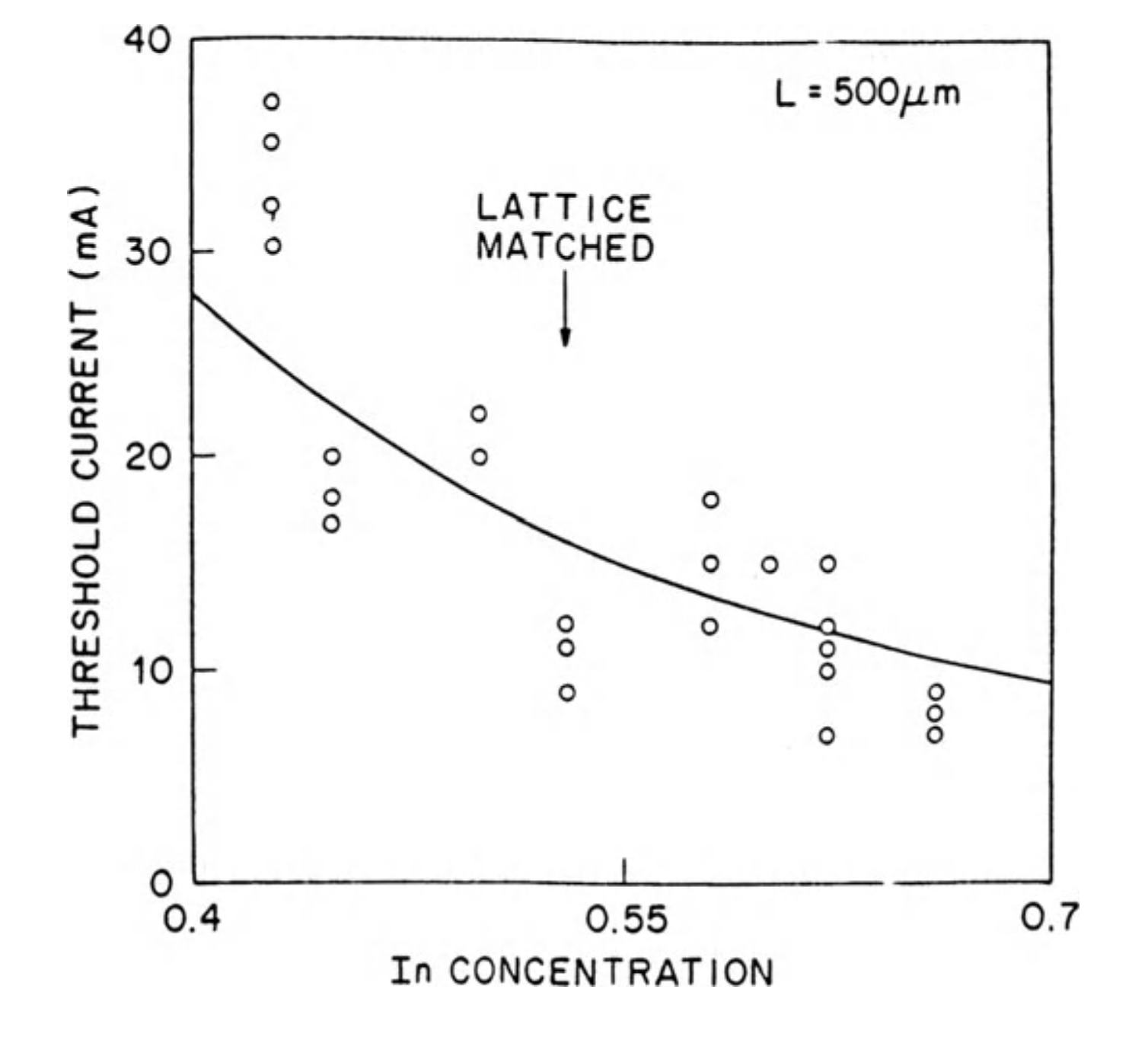Figure 9-26.  Measured threshold current plotted as a function of $$\text{In}$$ concentration $$x$$ for several InxGa1-xAs MQW lasers. The arrow indicates the lattice-matched composition ($$x=0.53$$).

Strained quantum well lasers fabricated using InxGa1-xAs layers grown over a GaAs substrate have been extensively studied.

The lattice constant of InAs is 60.6 pm and that of GaAs is 56.54 pm. The InxGa1-xAs alloy has a lattice constant, between these two values, and to a first approximation it can be assumed to vary linearly with $$x$$. Thus, an increase in the $$\text{In}$$ mole fraction $$x$$ increases the lattice mismatch relative to the GaAs substrate and therefore produces larger compressive strain on the active region.

A typical laser structure grown over the $$n$$-type GaAs substrate is shown in Figure 9-27 for this material system. It consists of an MQW active region with one to four InxGa1-xAs wells separated by GaAs barrier layers. The entire MQW structure is sandwiched between $$n$$- and $$p$$-type Al0.4Ga0.6As cladding layers, and the $$p$$-cladding layer is followed by a $$p$$-type GaAs contact layer.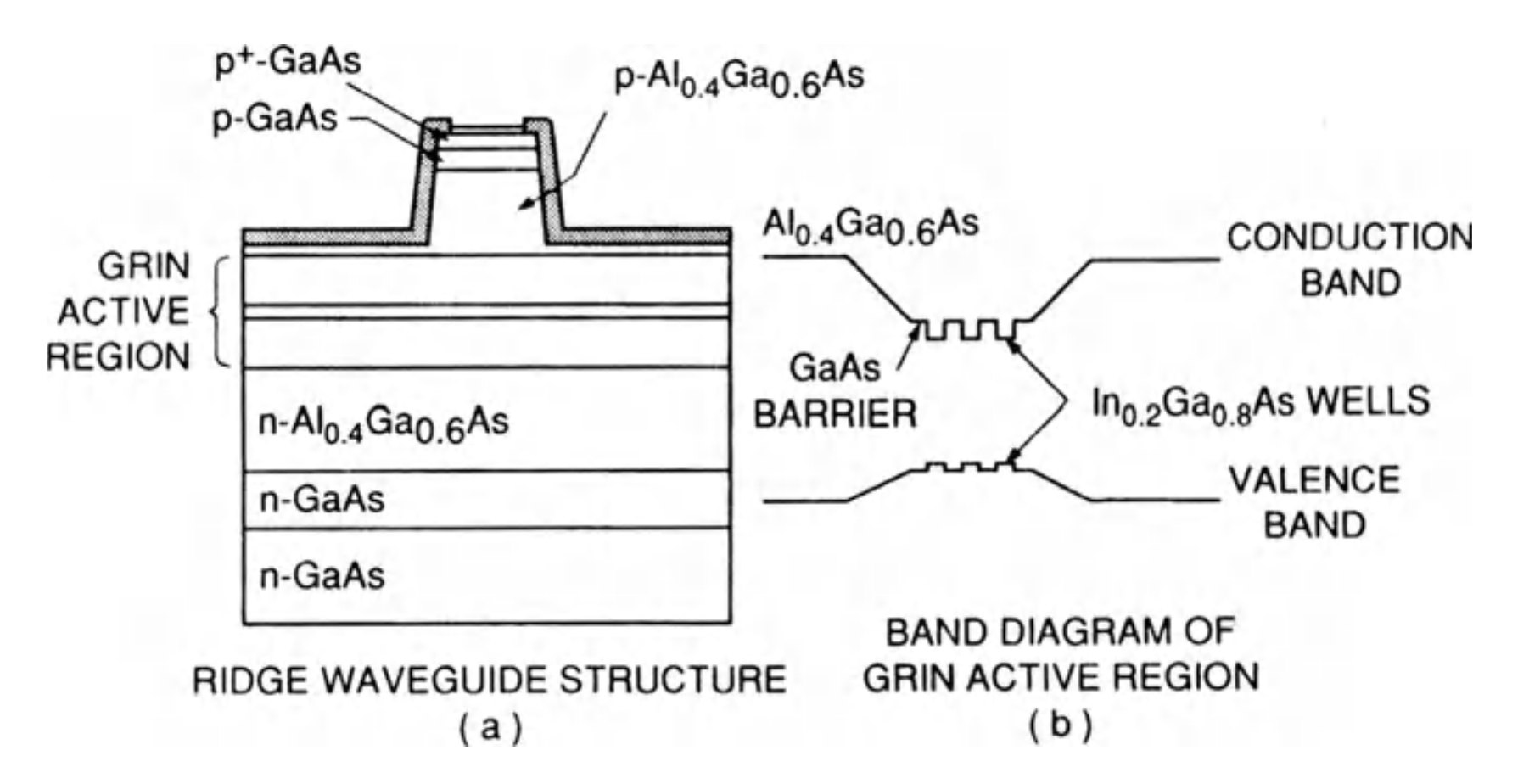Figure 9-27.  (a) Typical structure for an InxGa1-xAs MQW lasers. (b) Band diagram of graded-index active region.

Variations of the above structure with different cladding layers or large optical cavity designs have been reported. Emission wavelength depends on the $$\text{In}$$ composition, $$x$$. As $$x$$ increases, the emission wavelength increases (see Figure 9-28), and for $$x$$ larger than a certain value (typically ~ 0.25), the strain is too large to yield high-quality material.

For $$x\approx0.2$$, the emission wavelength is near 0.98 μm, a wavelength region of interest for pumping fiber amplifiers. A threshold current density as low as $$47\text{ A/cm}^2$$ has been reported for In0.2Ga0.8As-GaAs strained MQW lasers.

High-power lasers have been fabricated using an In0.2Ga0.8As-GaAs MQW active region. Single-mode output powers of greater than 200 mW have been demonstrated using a ridge-waveguide-type laser structure. CW output powers greater than 1 W have been demonstrated using a laser-array geometry. Aging test indicate high reliability for these strained quantum well lasers.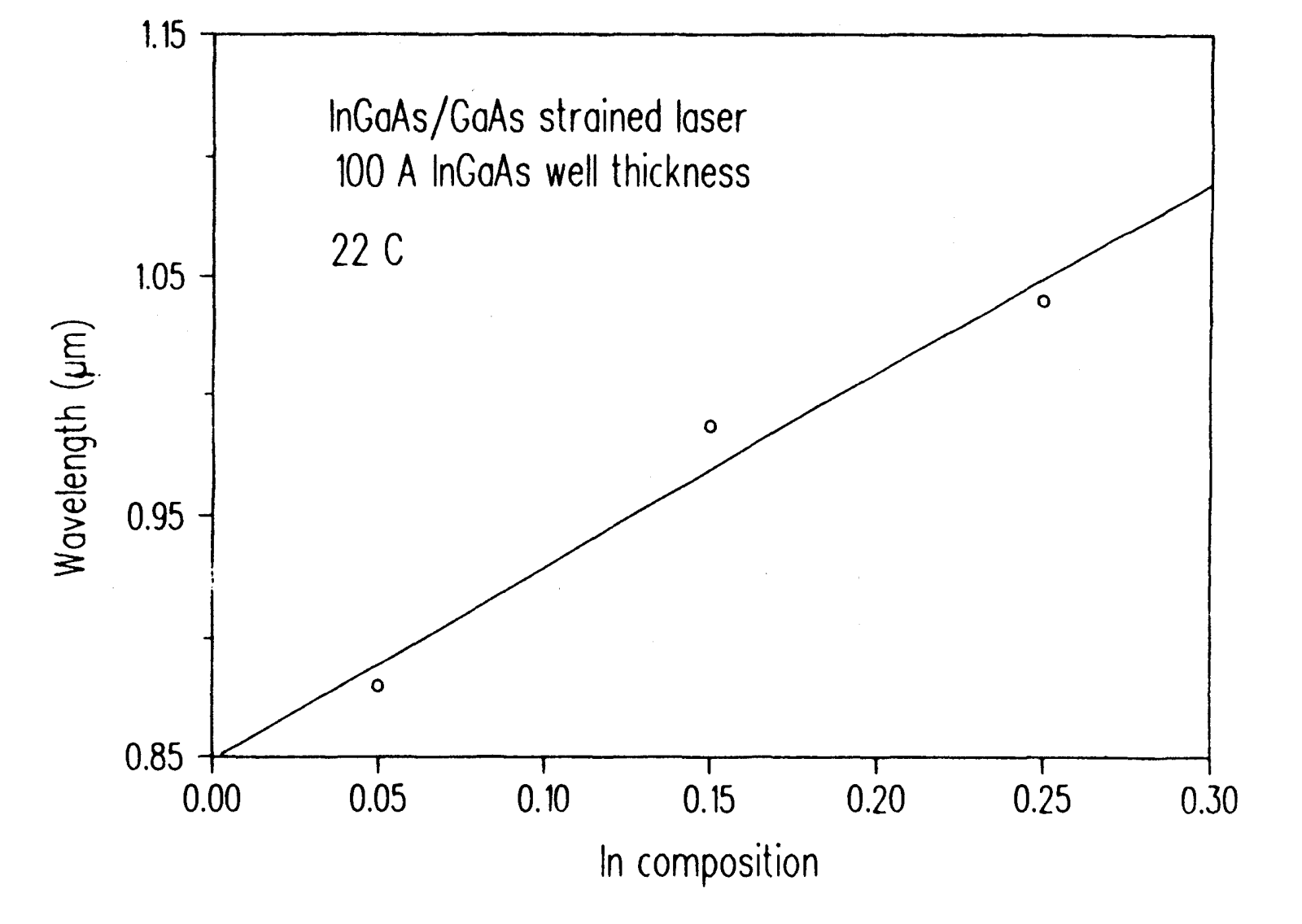Figure 9-28.  Measured wavelength plotted as a function of $$\text{In}$$ concentration $$x$$ for several  InxGa1-xAs MQW lasers.

The frequency chirp of strained and unstrained QW lases has been measured. Strained QW lasers (InGaAs-GaAs) exhibit the lowest chirp (or dynamic line width) under modulation. The lower chirp of strained QW lasers is consistent with a small line-width enhancement factor measured in such devices.

The correlation between the measured chirp and line-width enhancement factor for regular double-heterostructure, strained and unstrained QW lasers is shown in Table 9-1.

The high efficiency, high power and low chirp of strained and unstrained QW lasers make these devices attractive for lightwave transmission applications.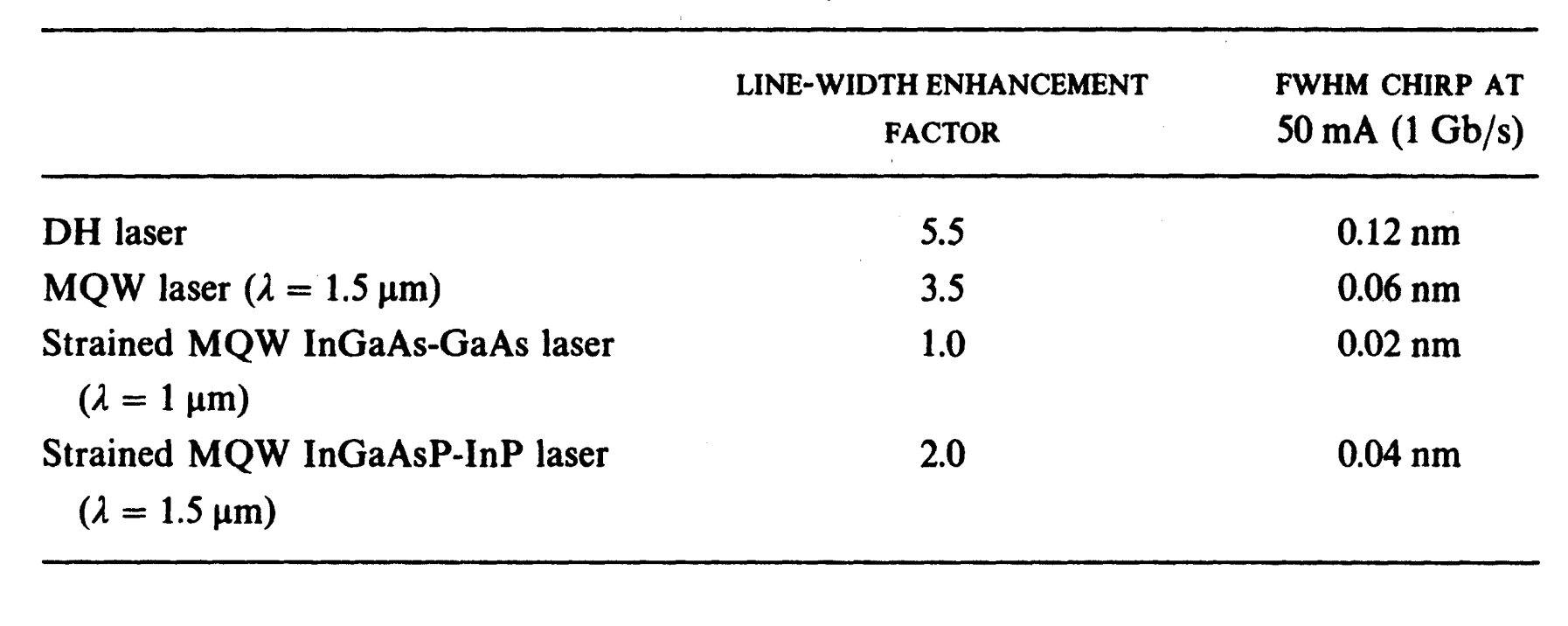Table 9-1.  Correlation between the measured chirp and line-width enhancement factor for regular double-heterostructure, strained and unstrained QW lasers.

The next tutorial discusses in detail of vertical-cavity surface-emitting lasers (VCSEL).